# RD Sharma Solutions for Class 10 Maths Chapter 7 Statistics

## RD Sharma Solutions Class 10 Maths Chapter 7 – Free PDF Download

RD Sharma Solutions for Class 10 Maths Chapter 7 – Statistics are provided here for students to access easily. If students are looking for a last-minute reference source, then RD Sharma Solutions is like the best friend who brushes up on all the important concepts and their formulas. Furthermore, this is an essential tool to qualify for the exams with high marks. It is created by our team of experts at BYJU’S, keeping in mind the latest CBSE norms and also in simple language with suitable explanations.

One of the interesting chapters of RD Sharma Class 10 Statistics, it is the 7th chapter and comprises six exercises. Students can access the answers to the exercises included in RD Sharma Solutions for Class 10 here. The chapter basically deals with the techniques for finding the mean, median and mode of grouped data. It also includes the concept of a cumulative frequency graph of a frequency distribution.

## Download the PDF of RD Sharma Solutions for Class 10 Maths Chapter 7 Statistics Here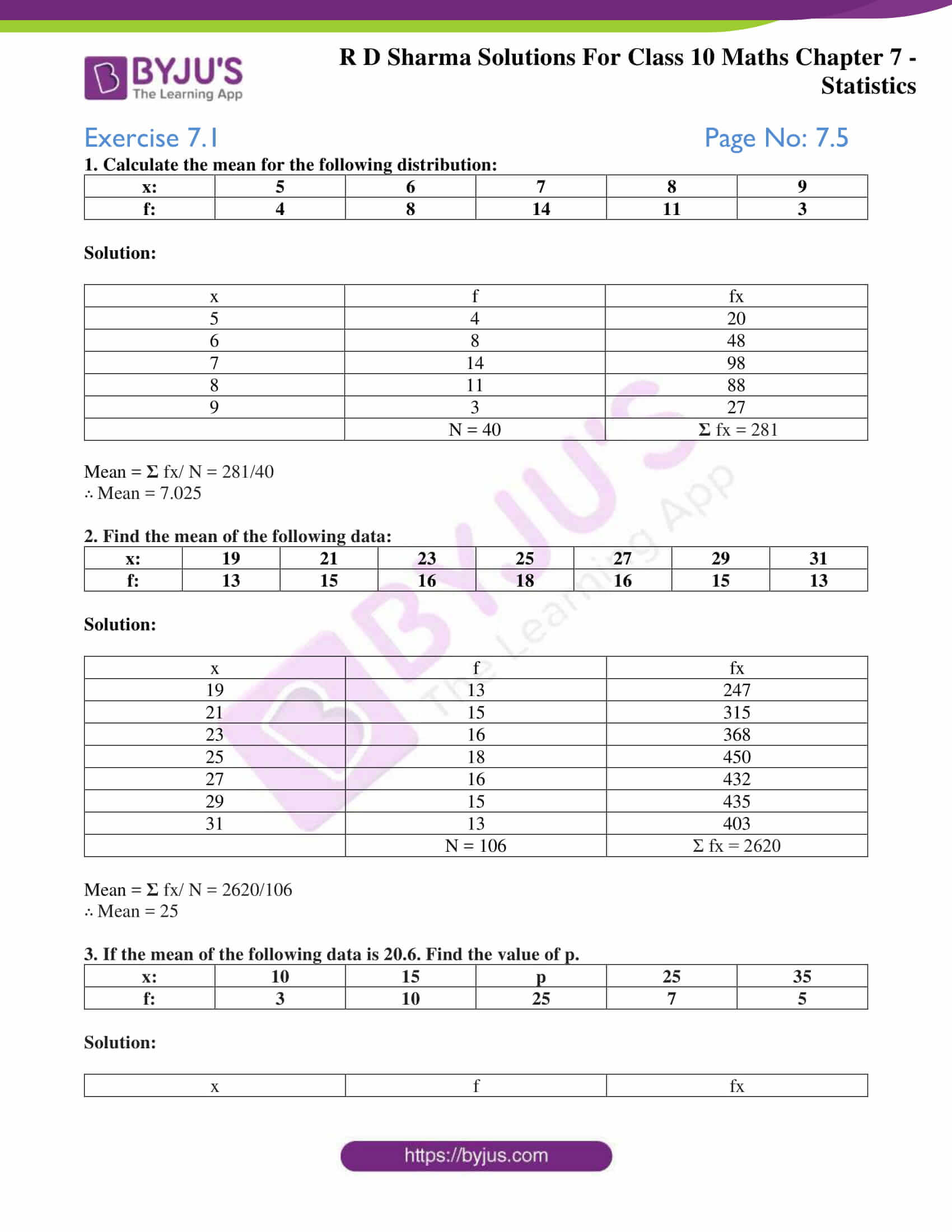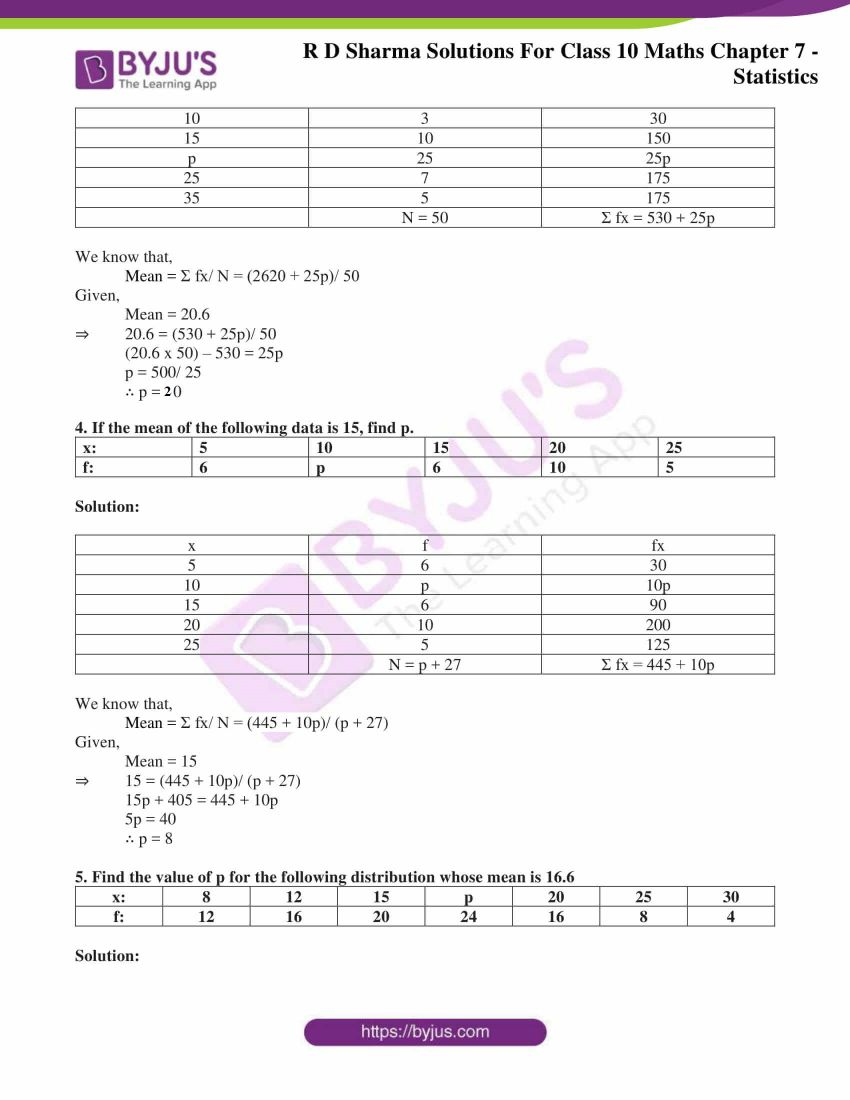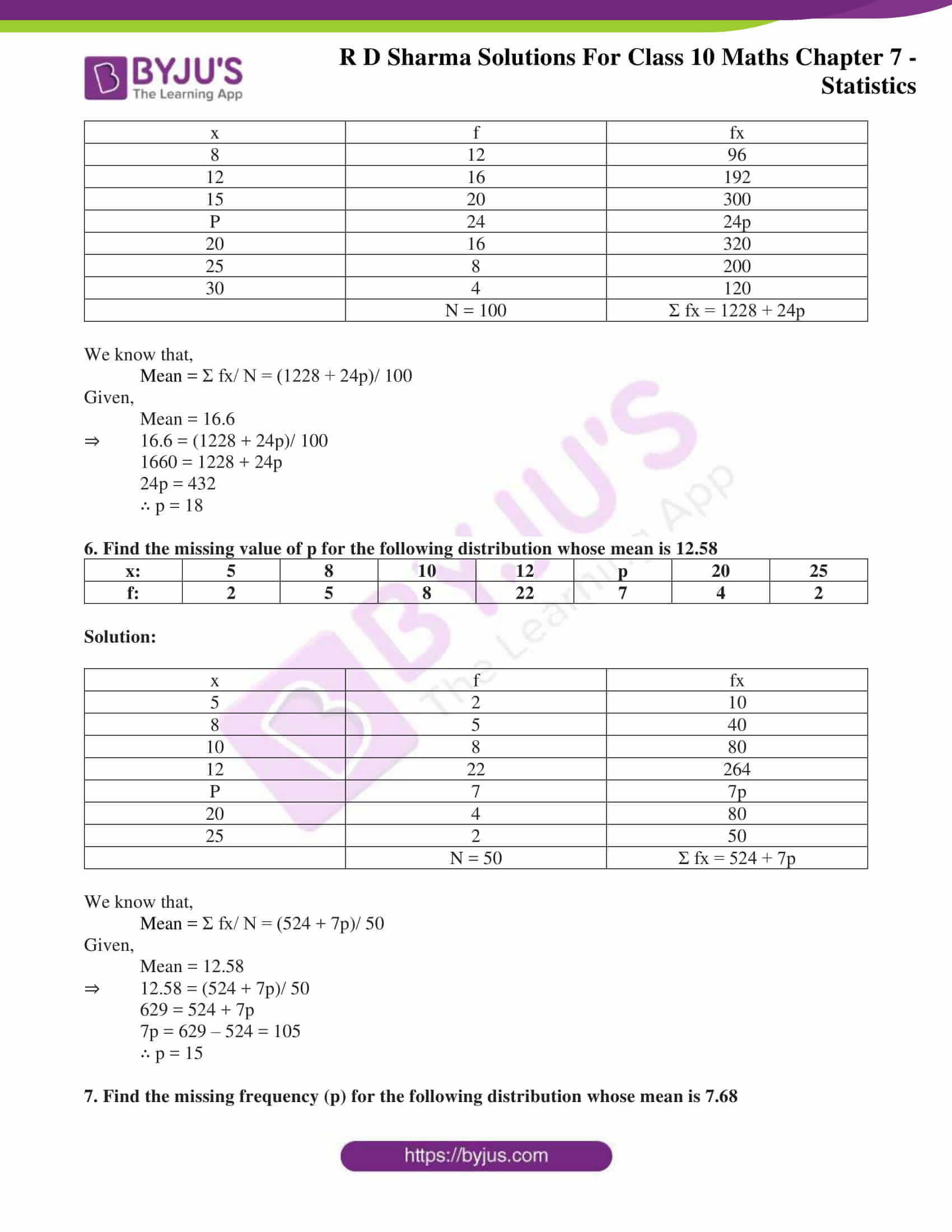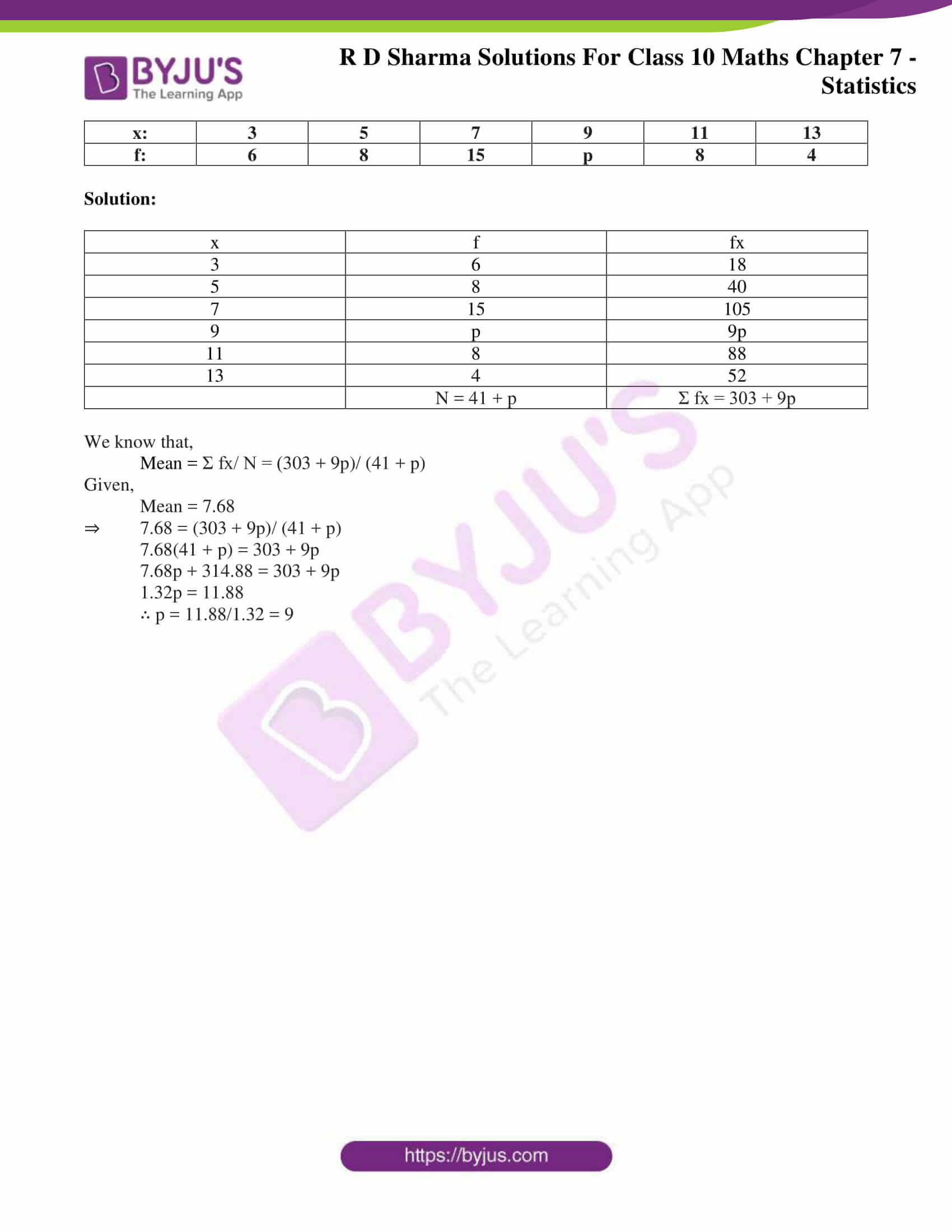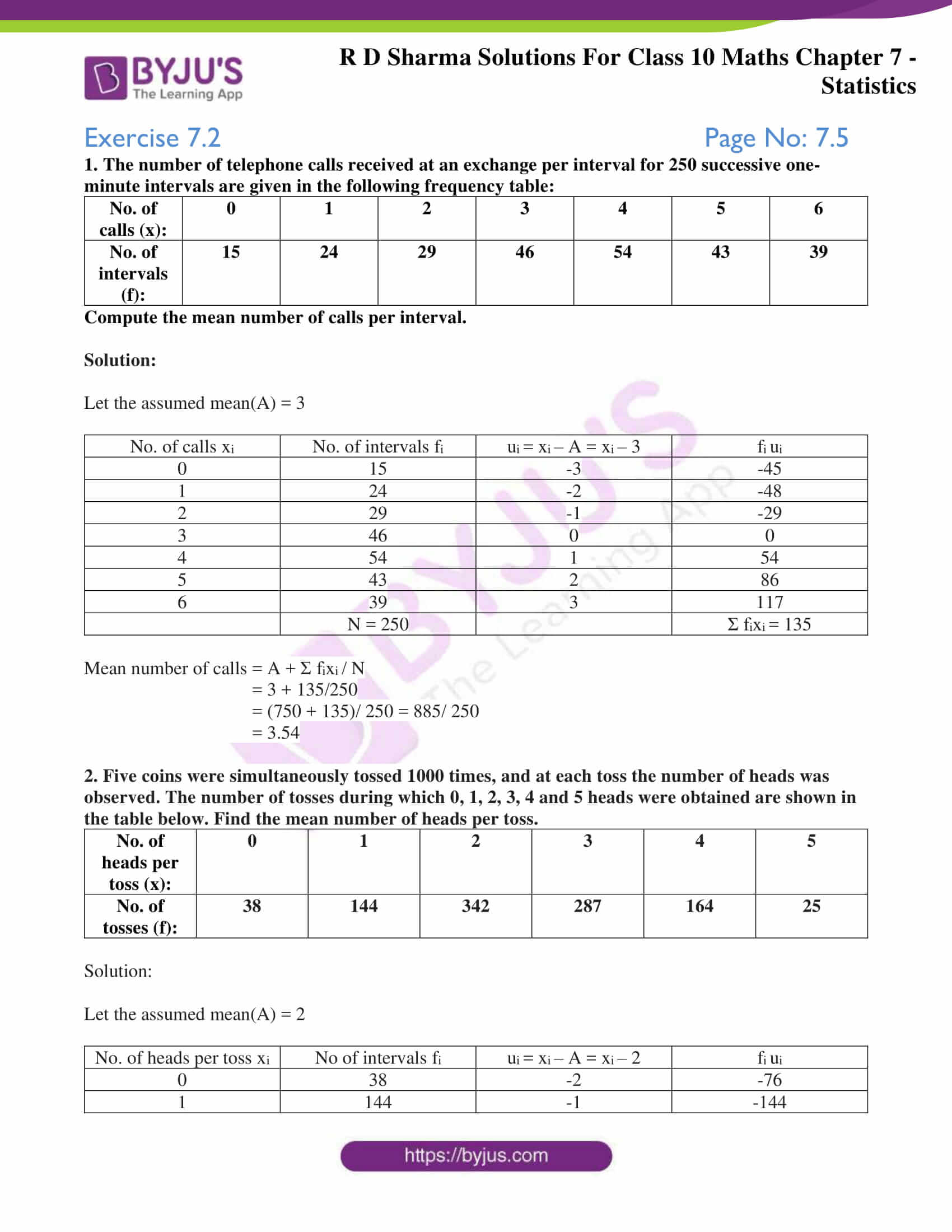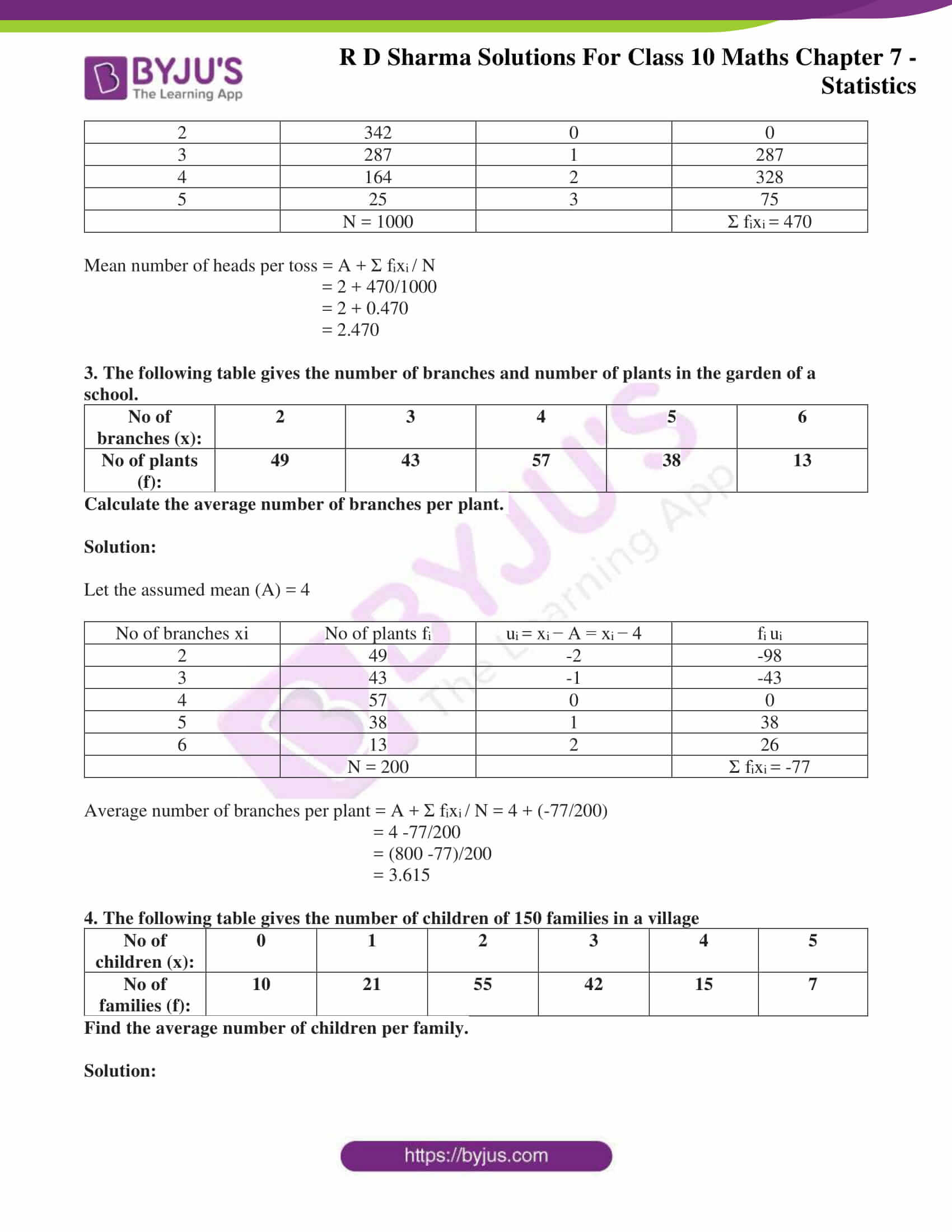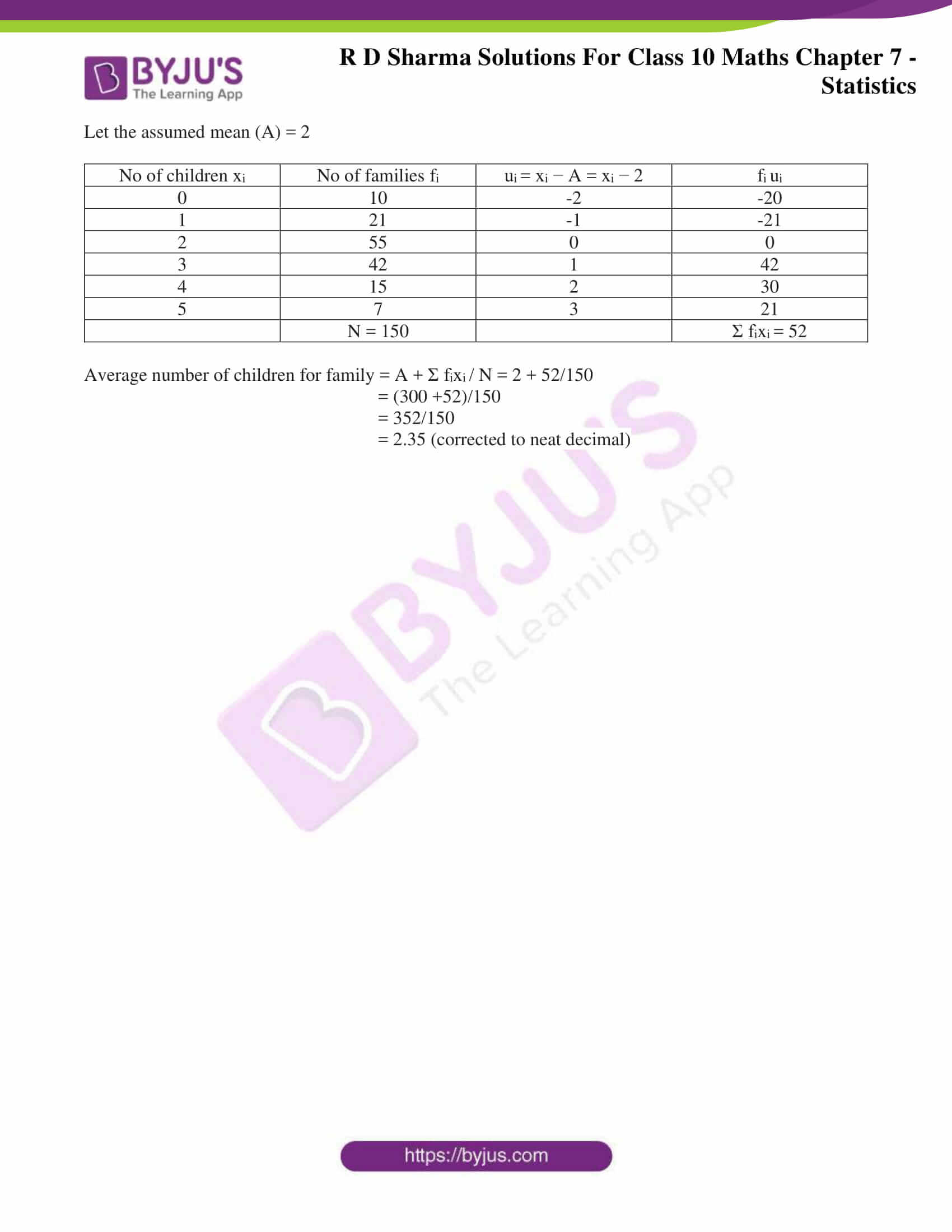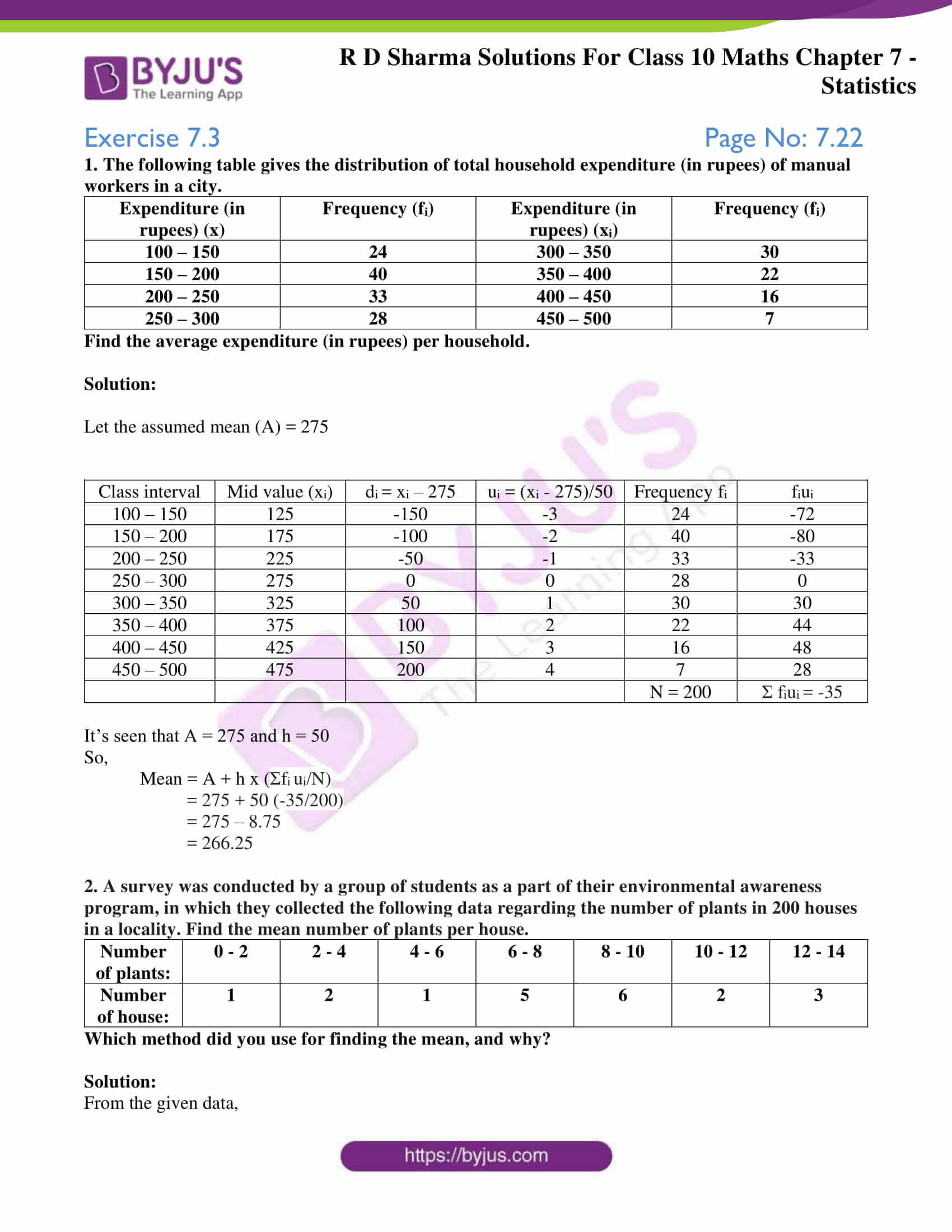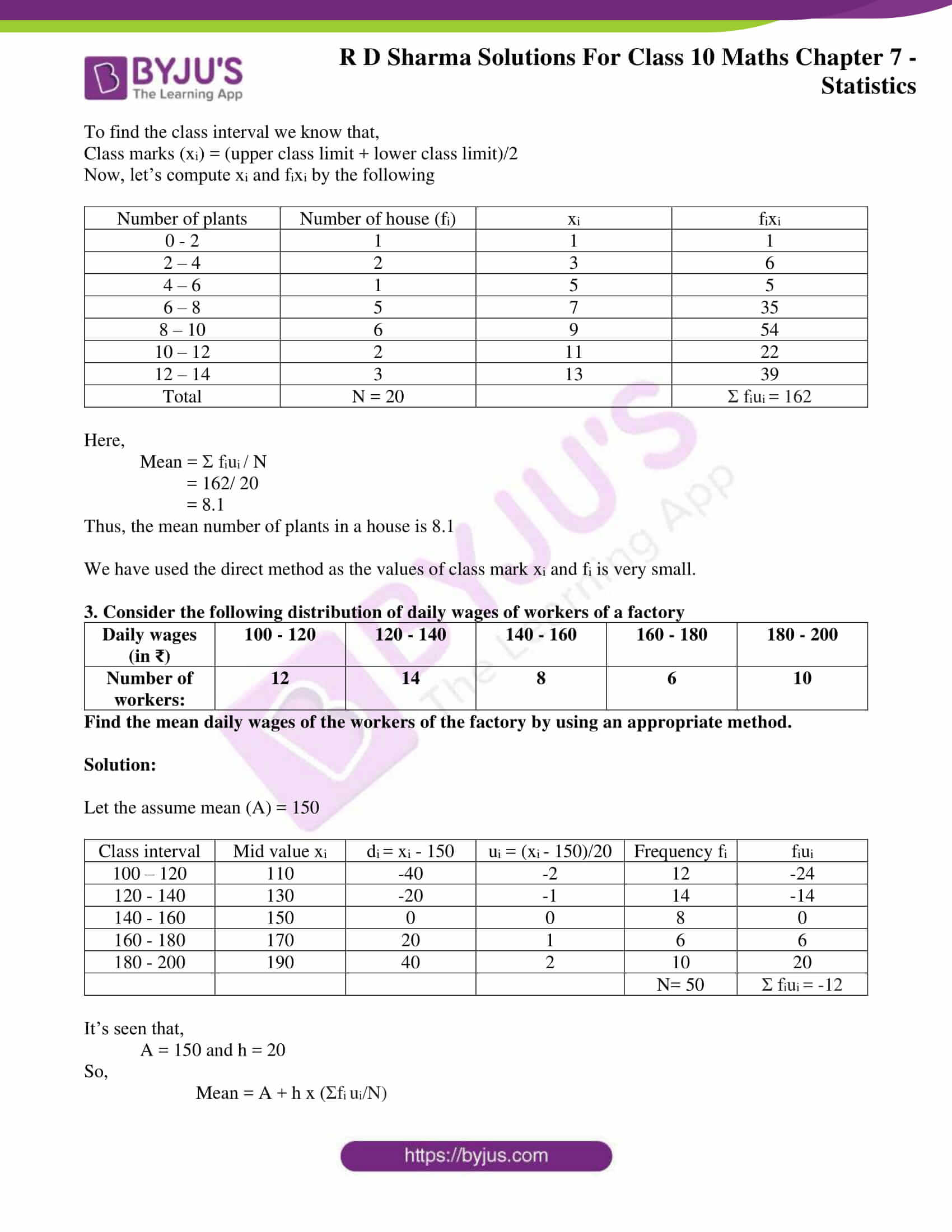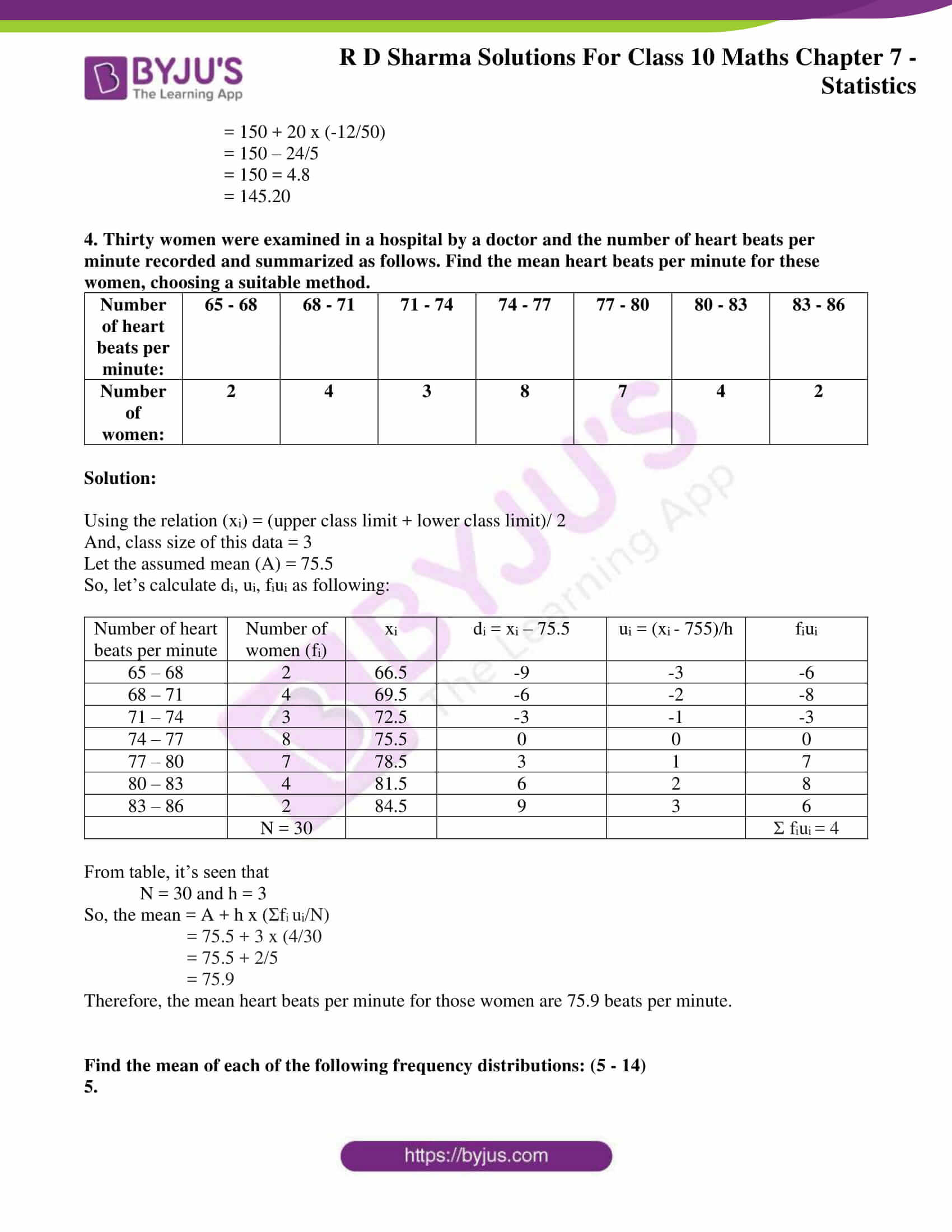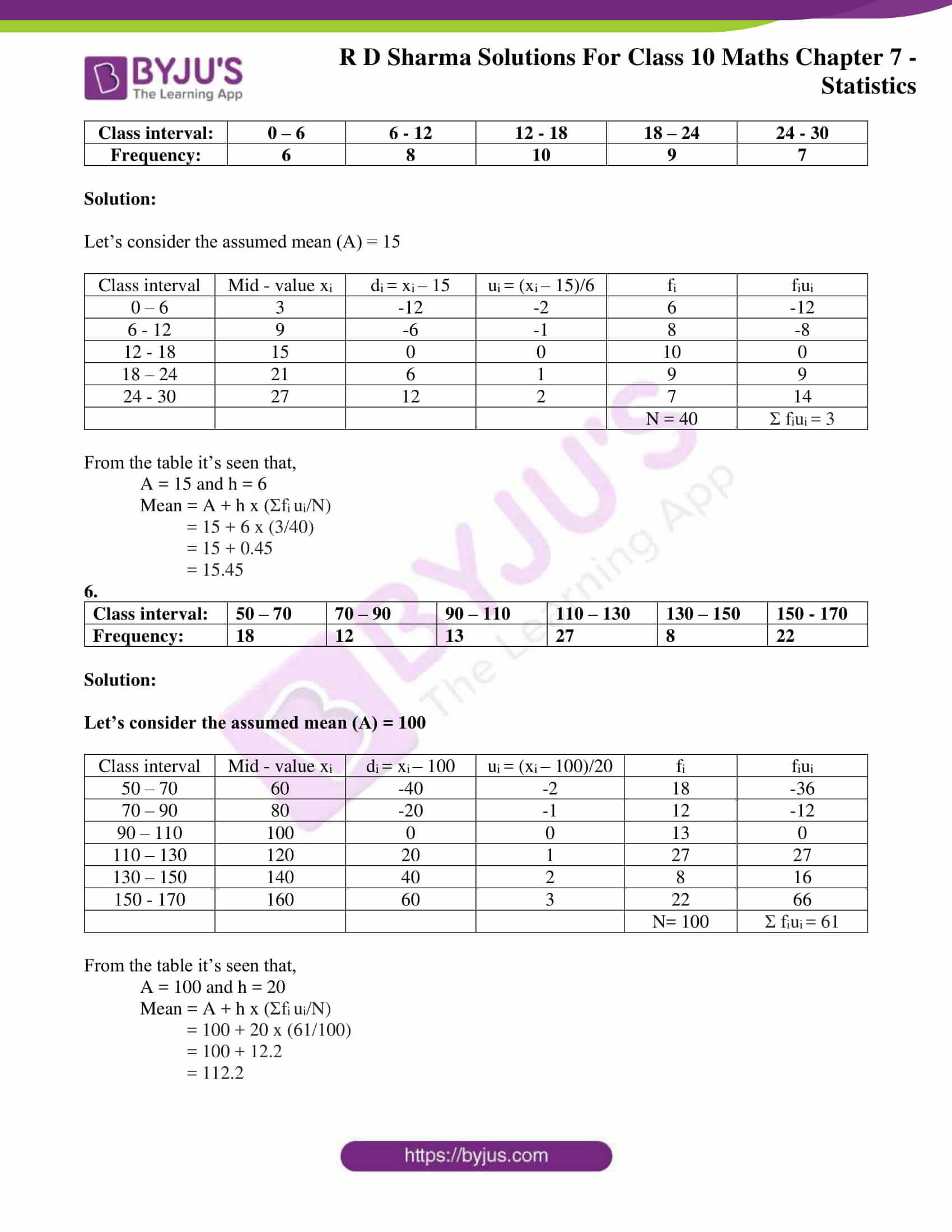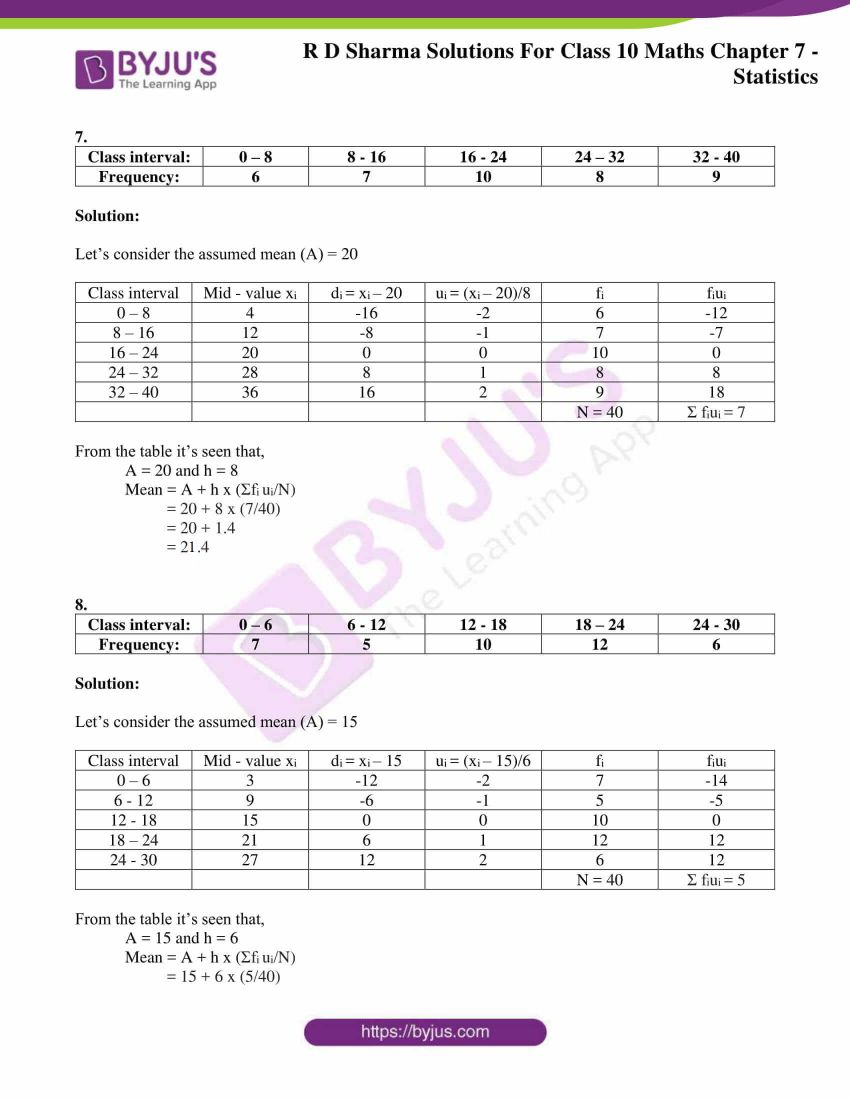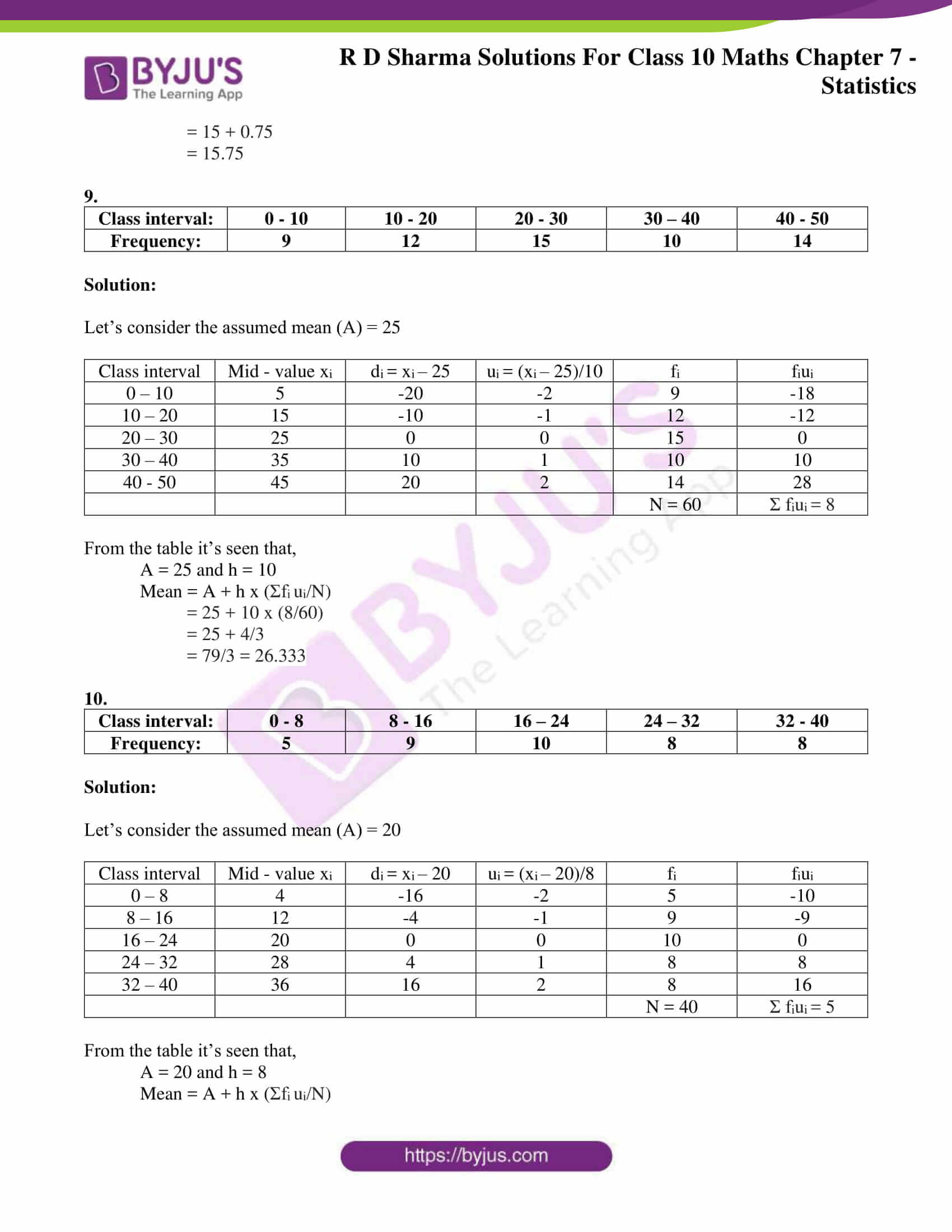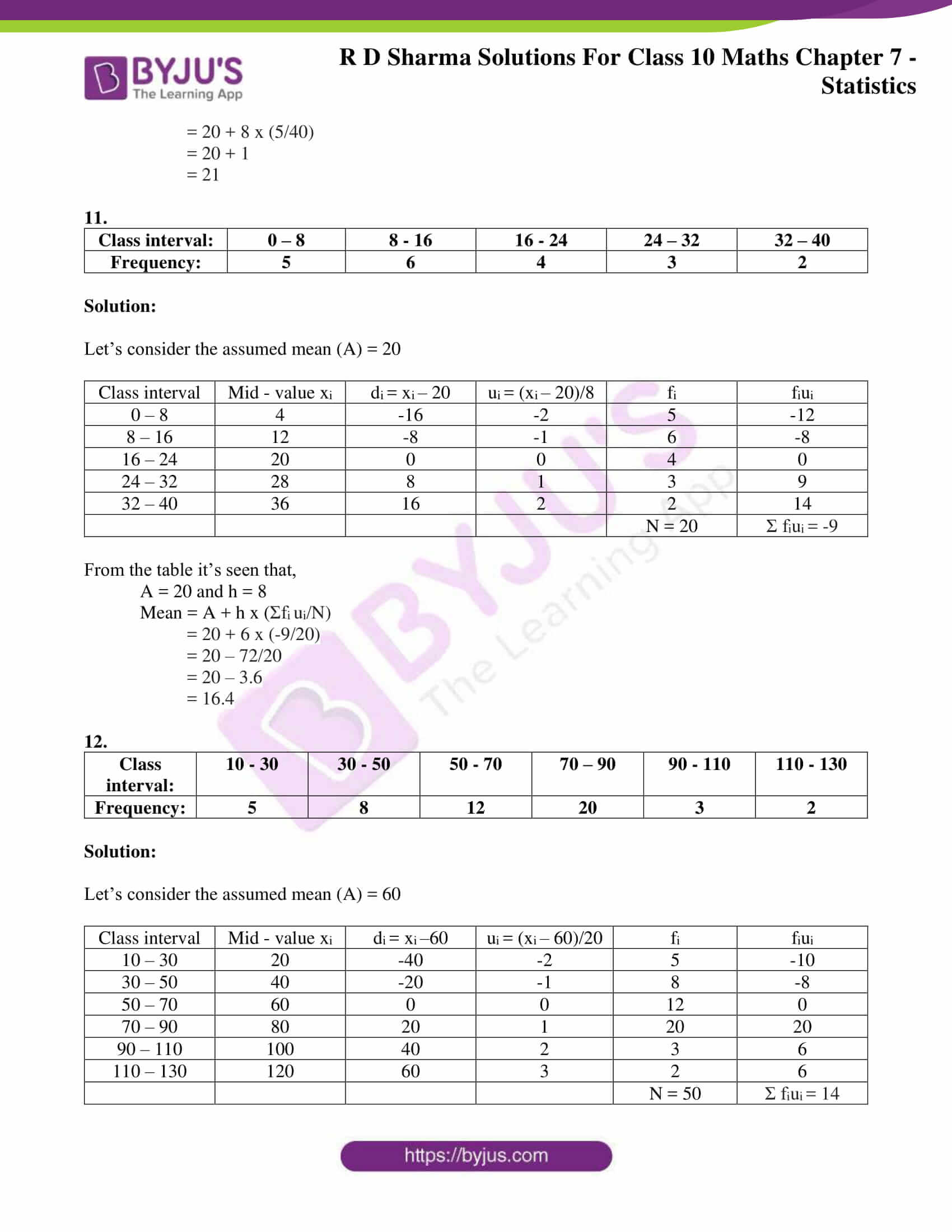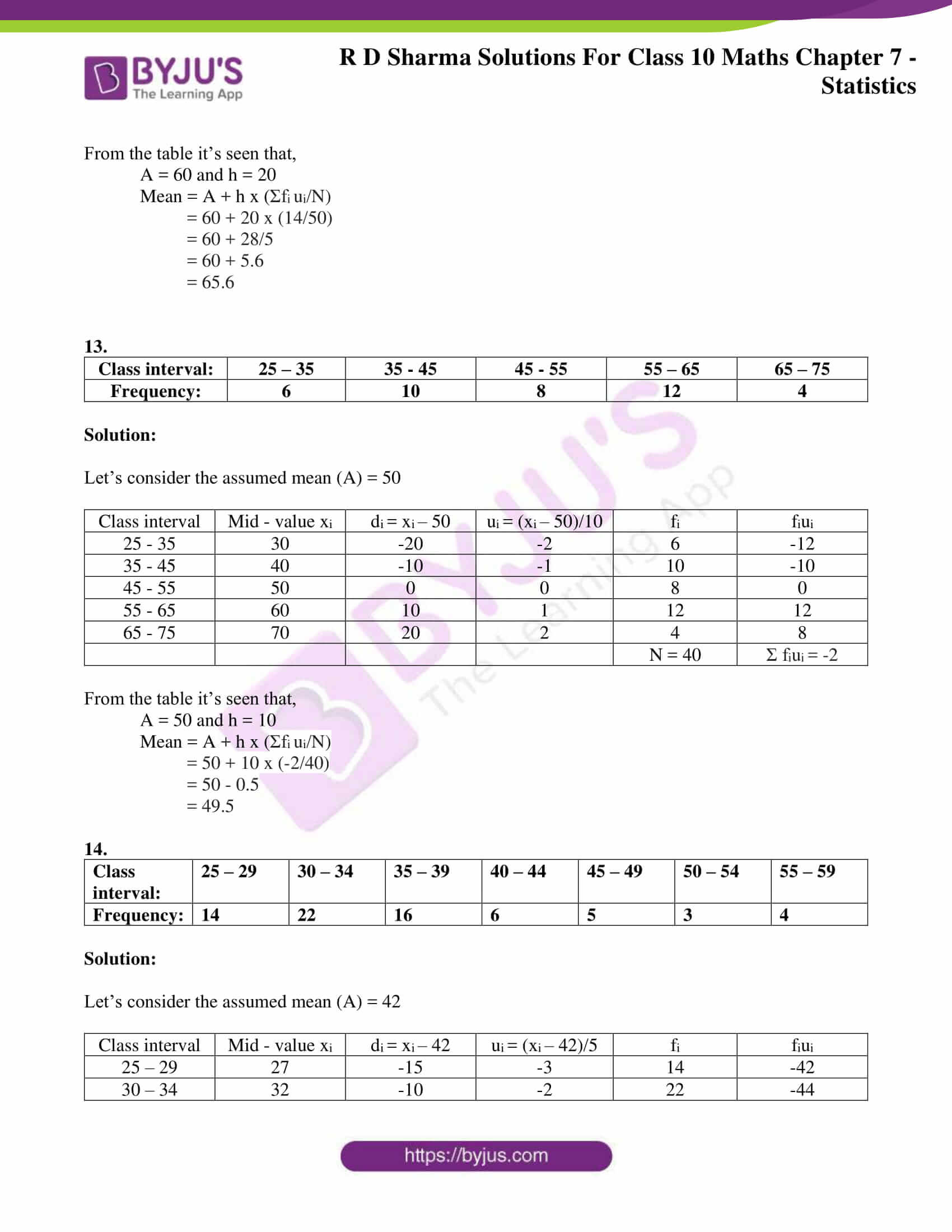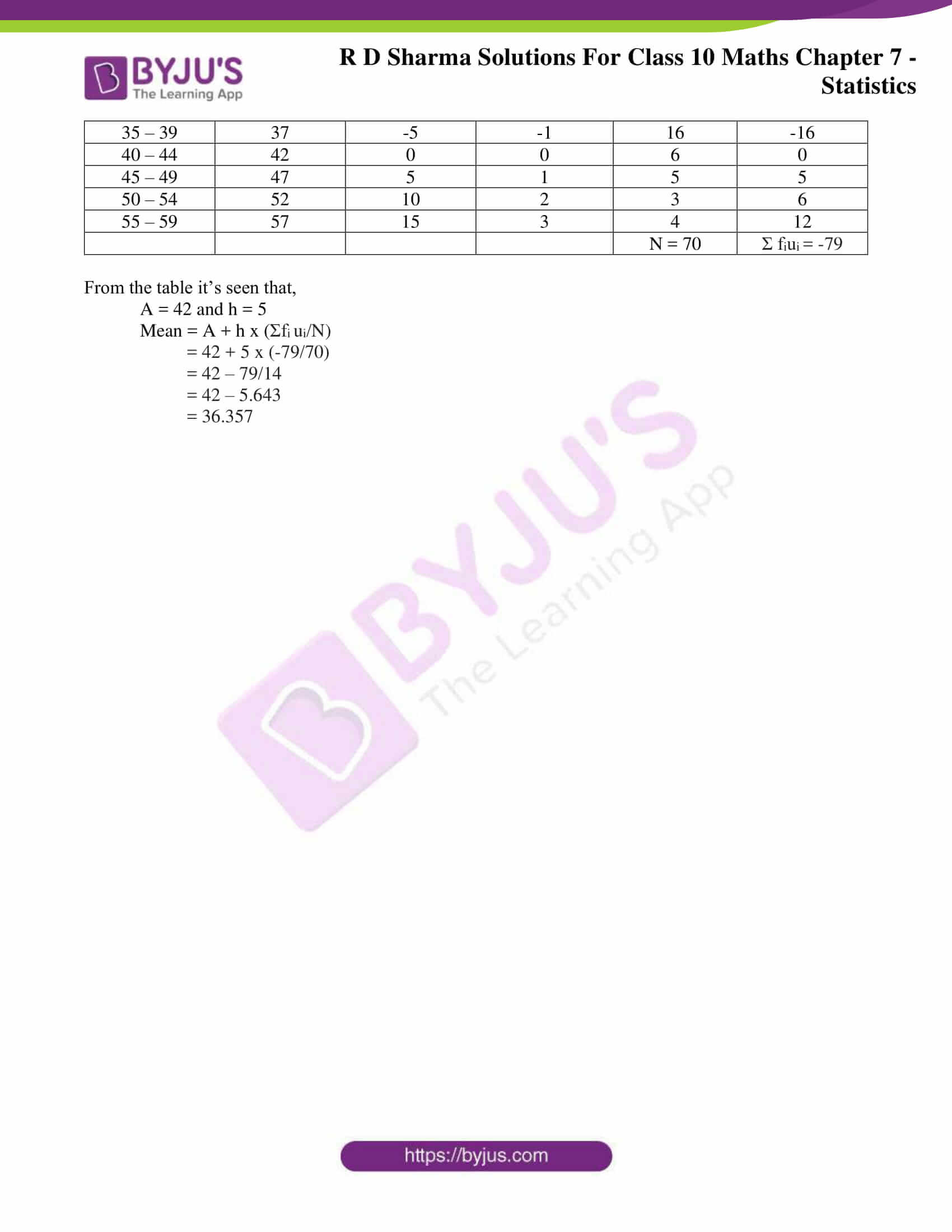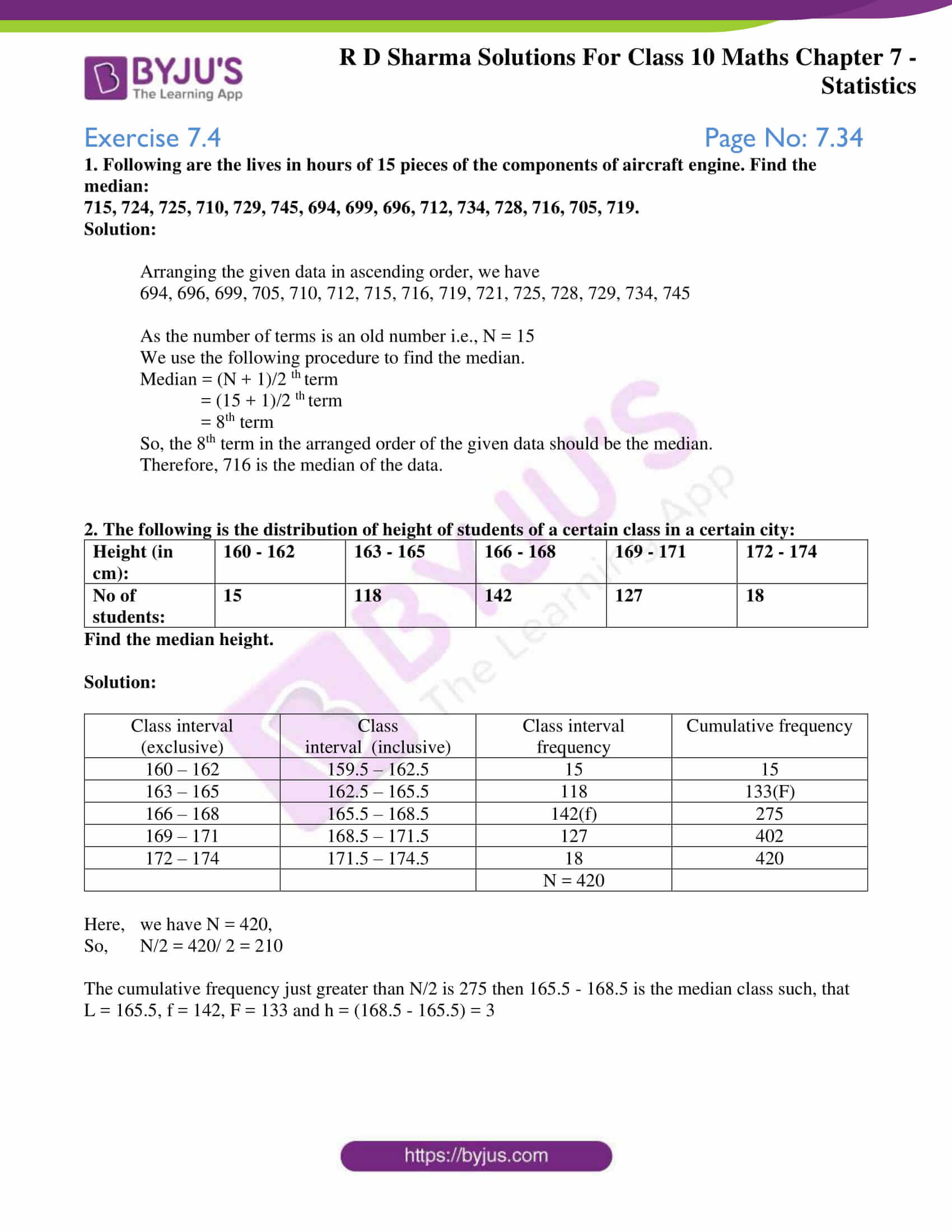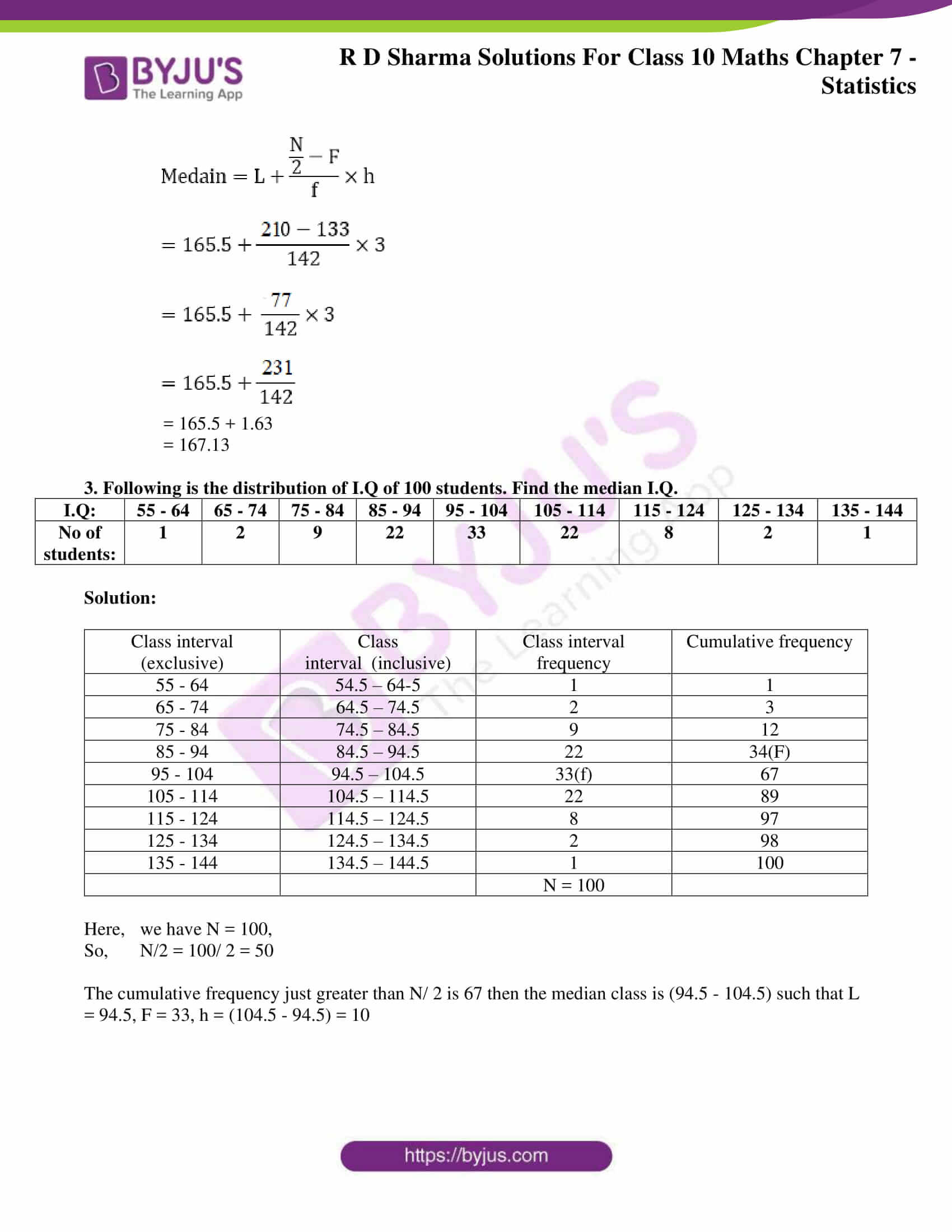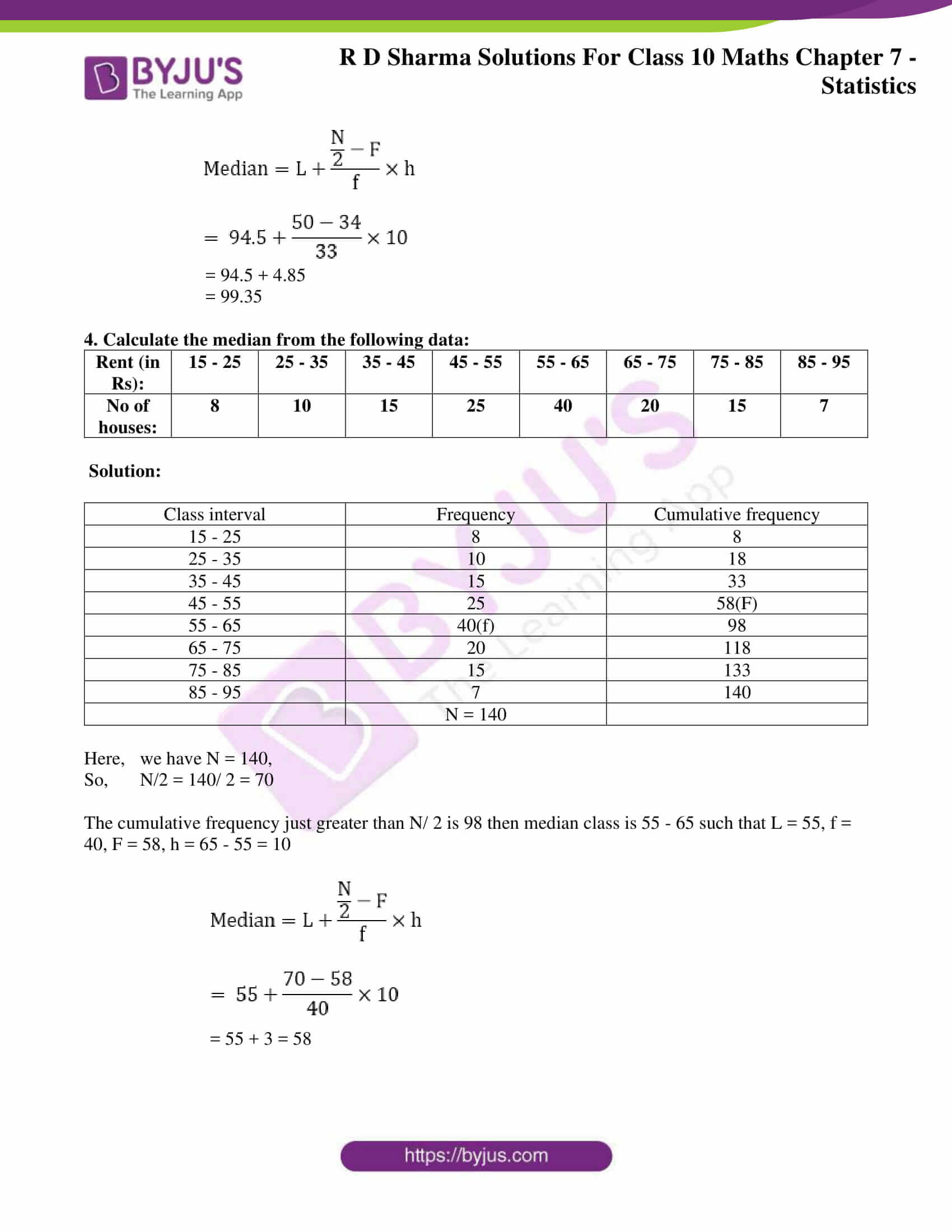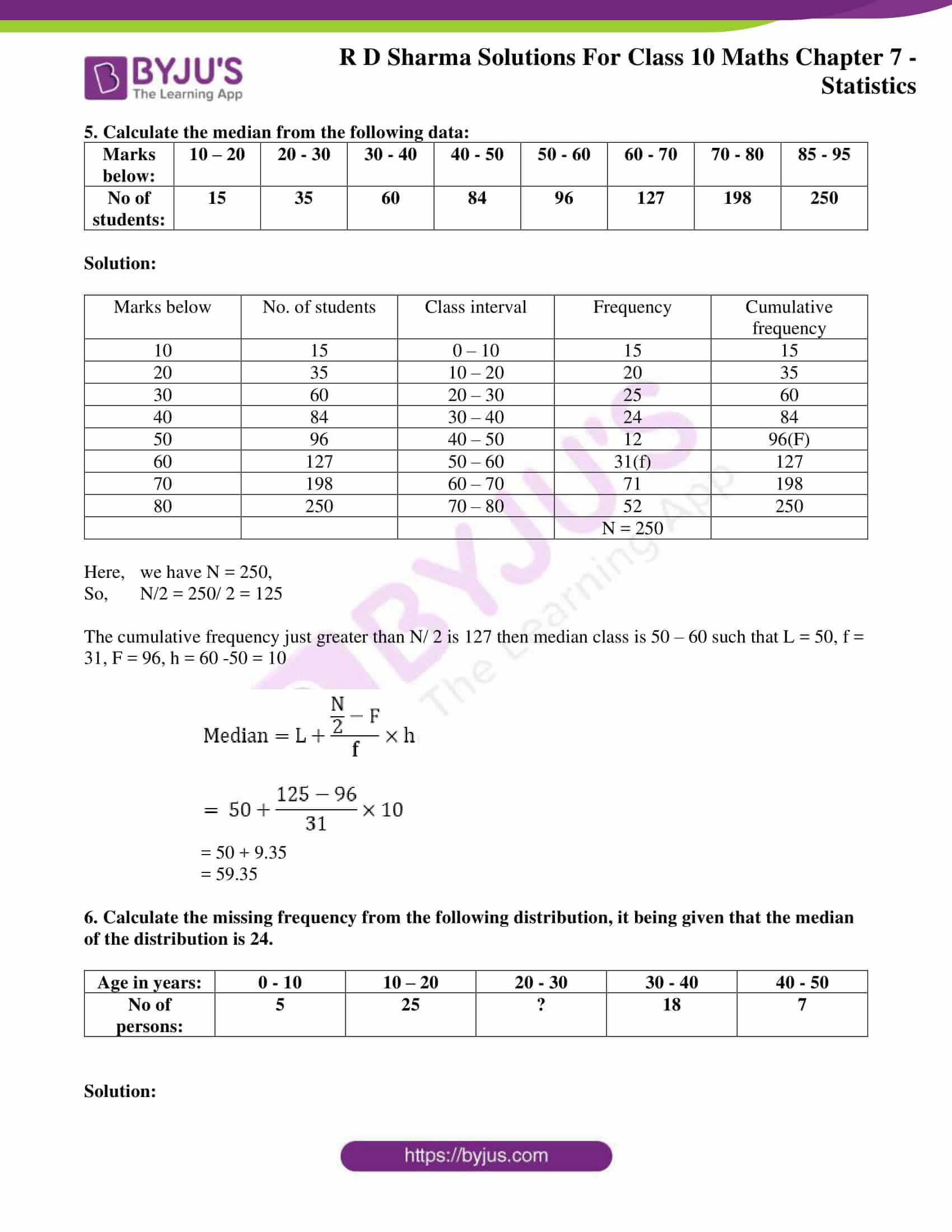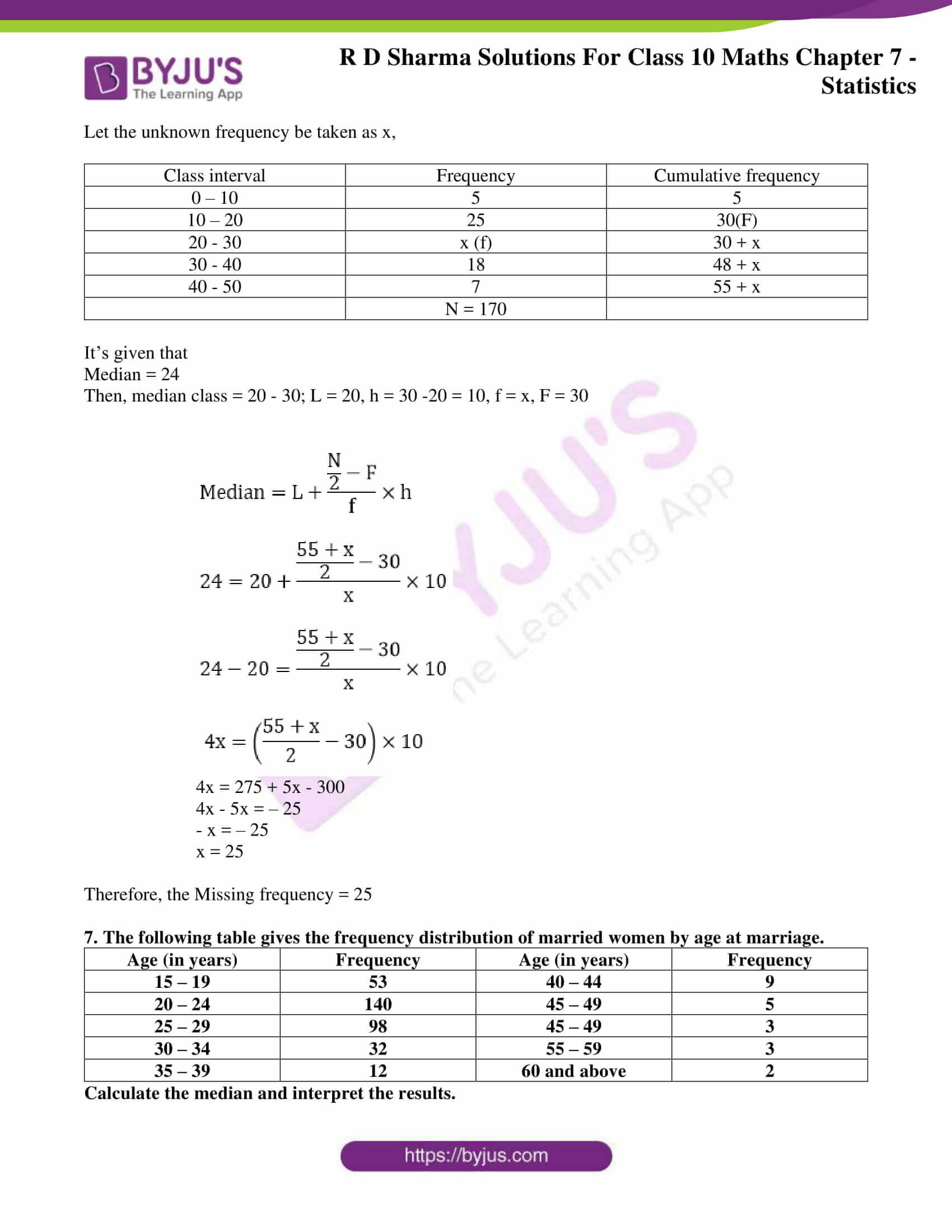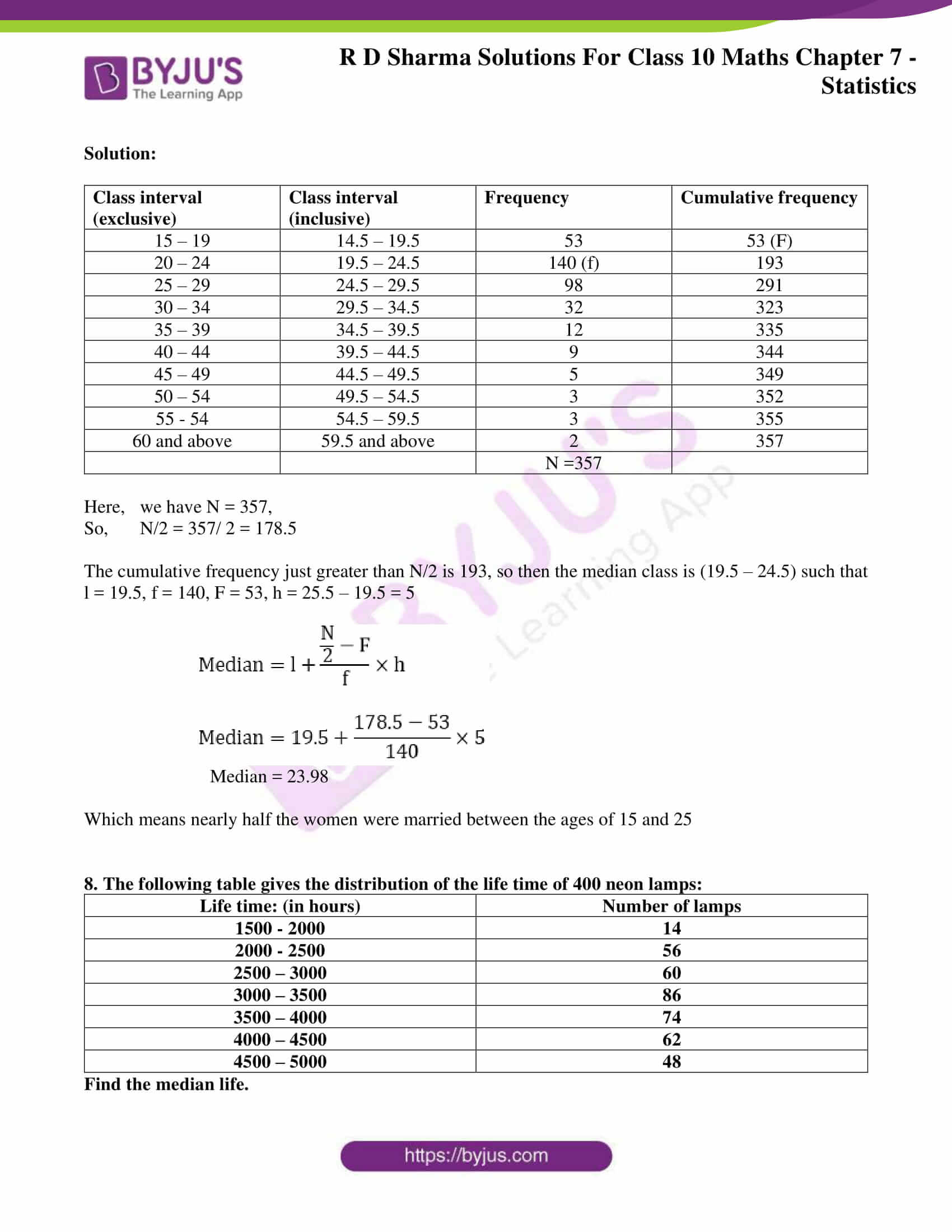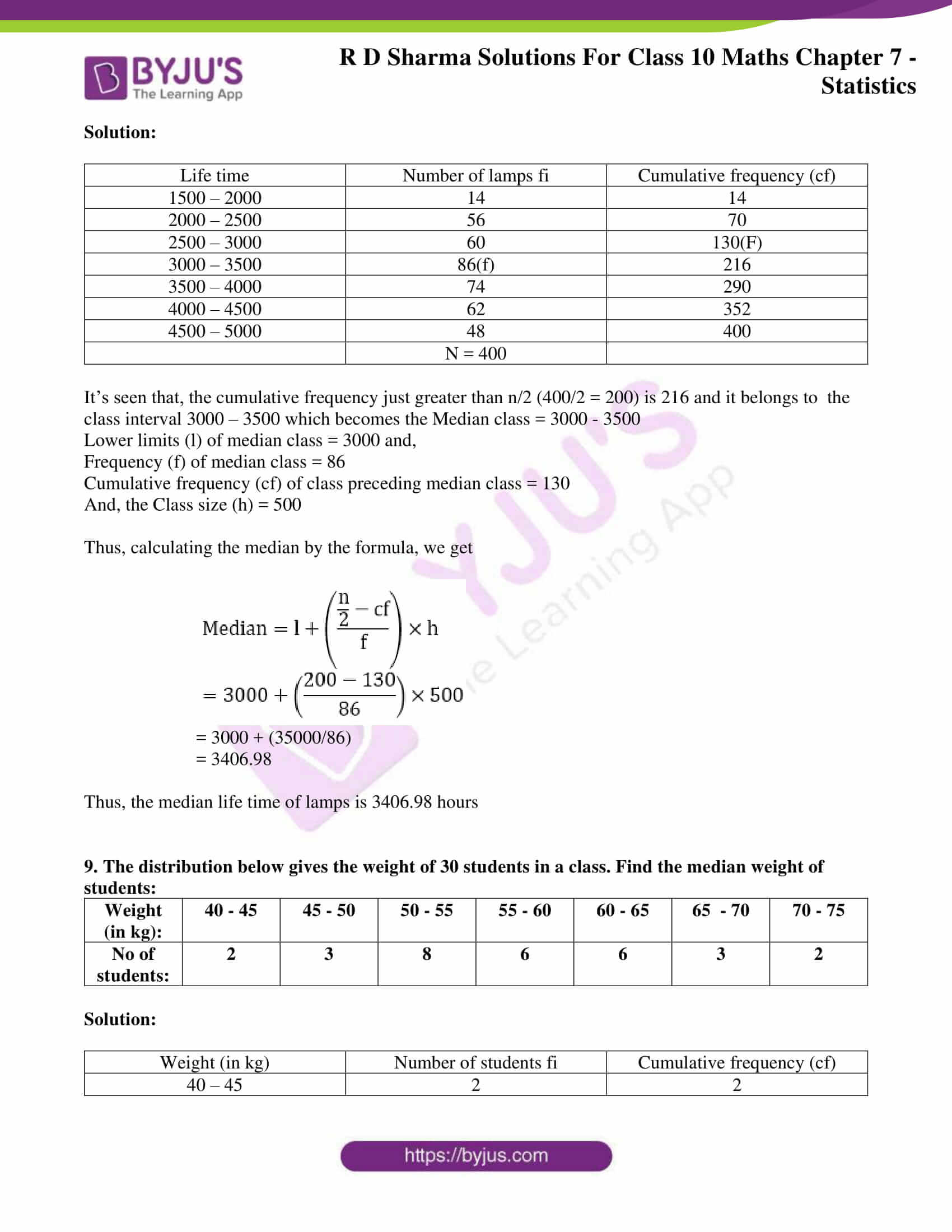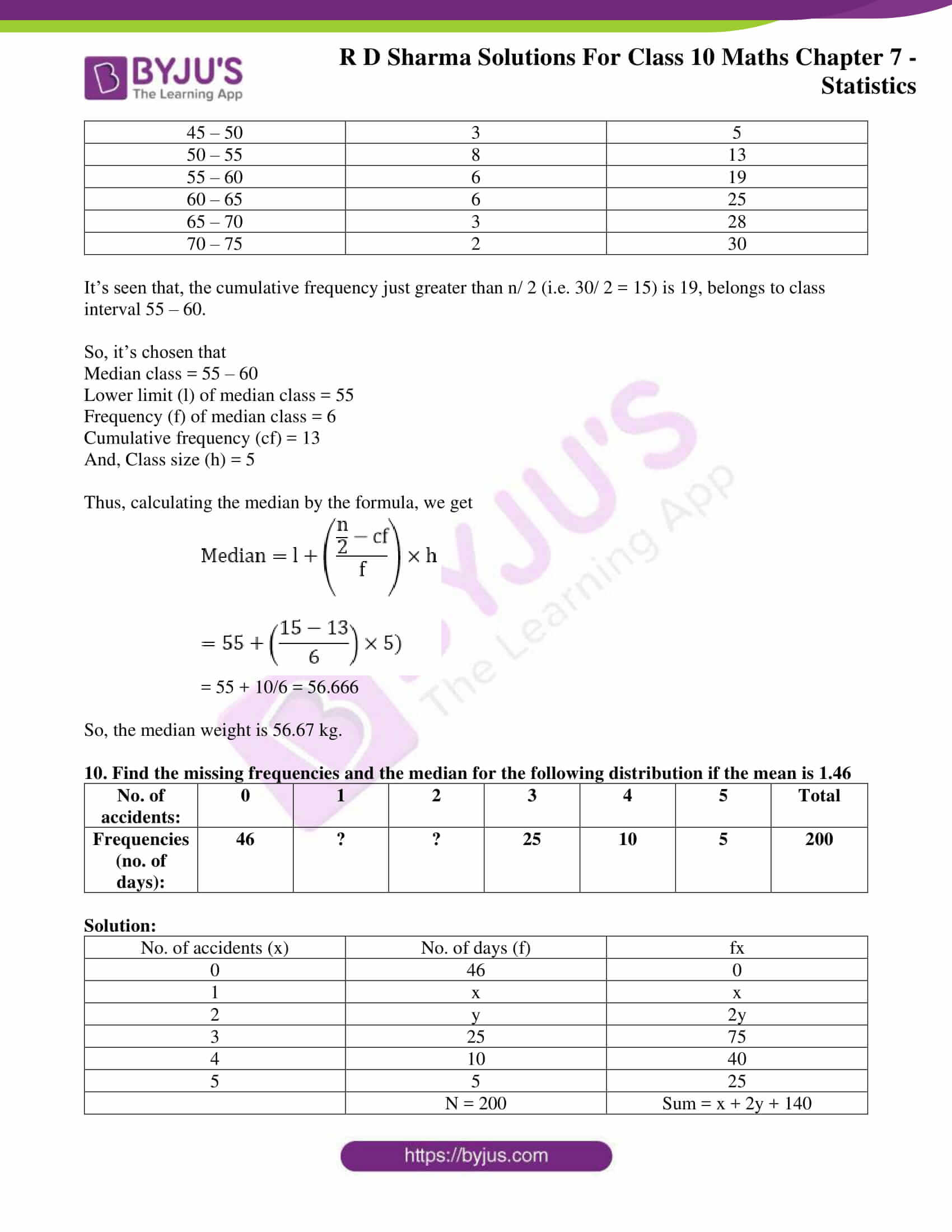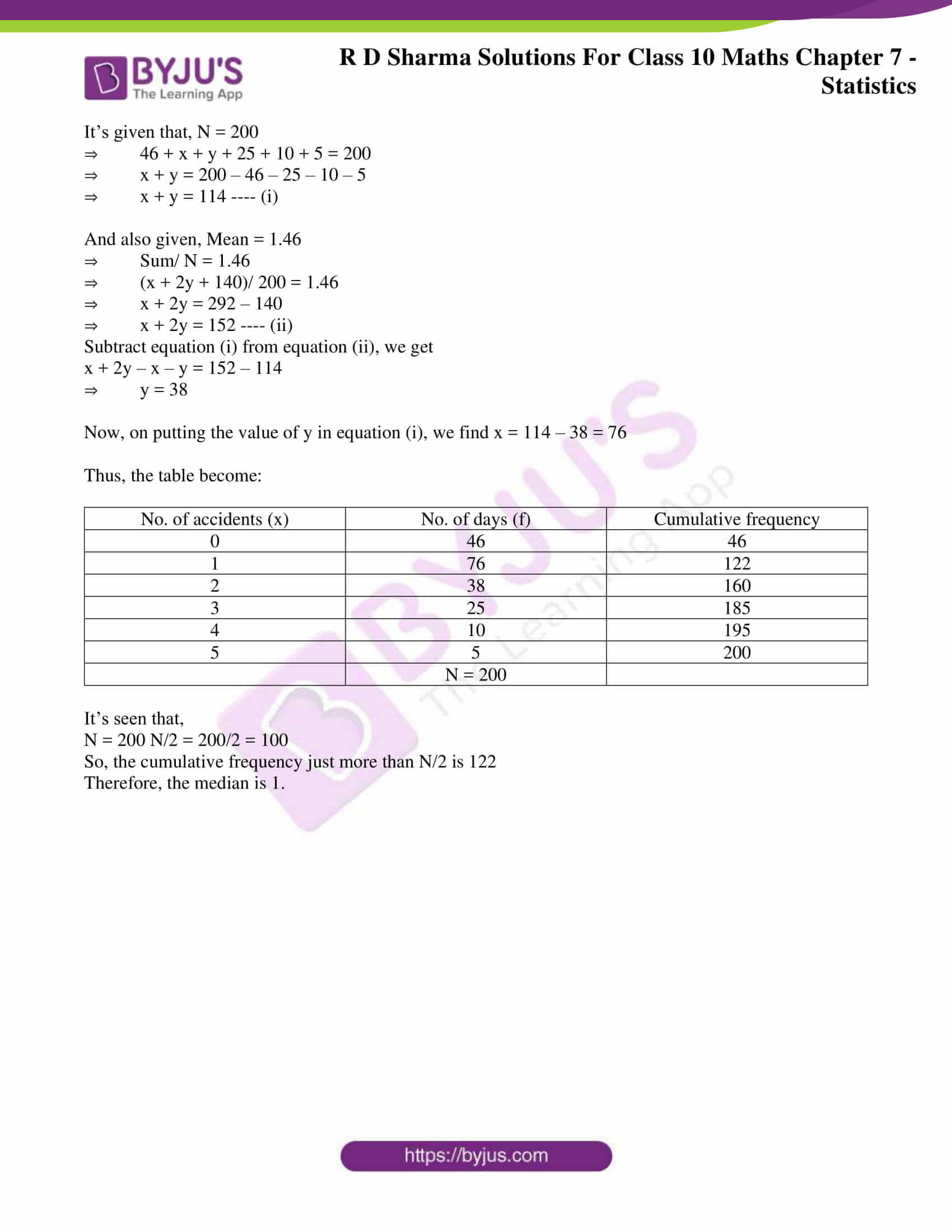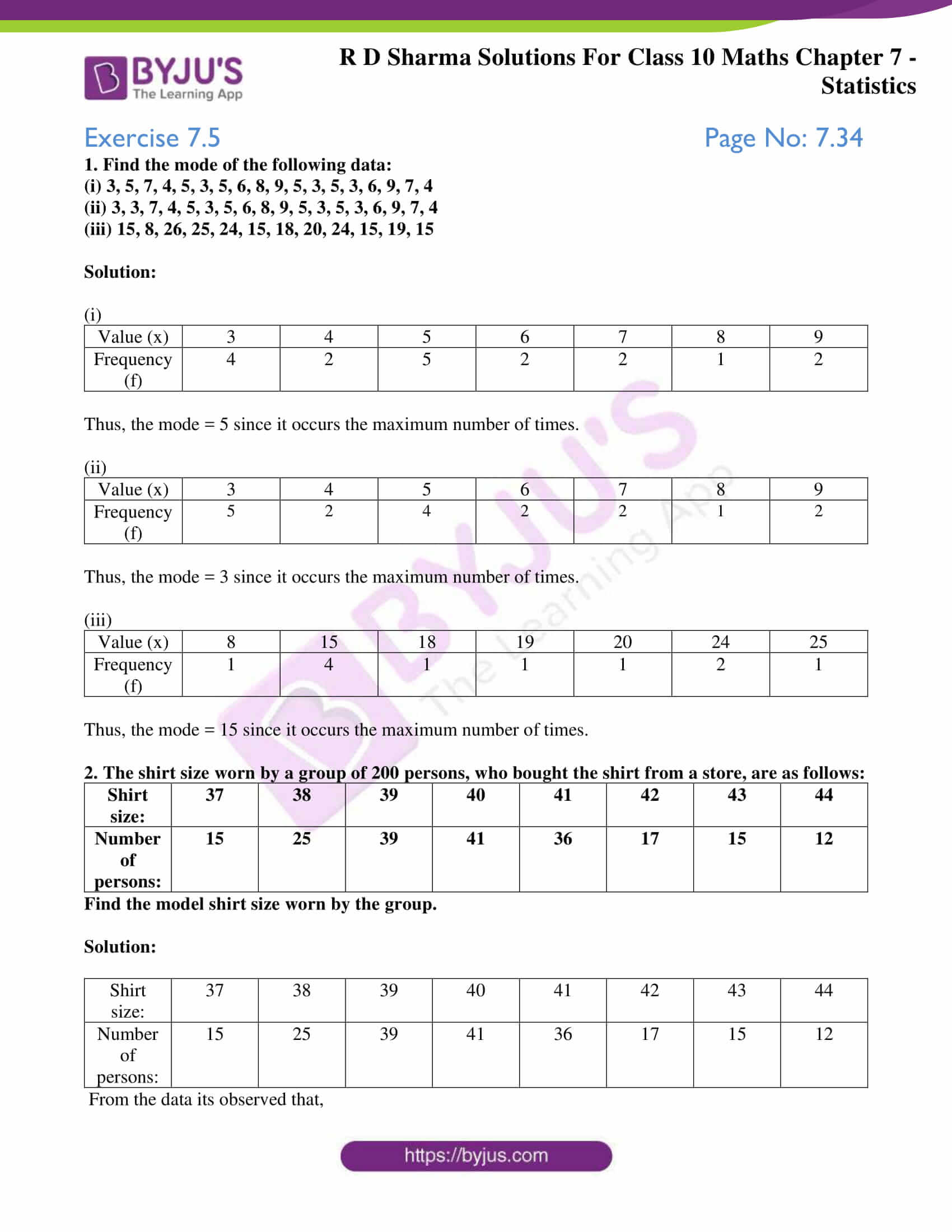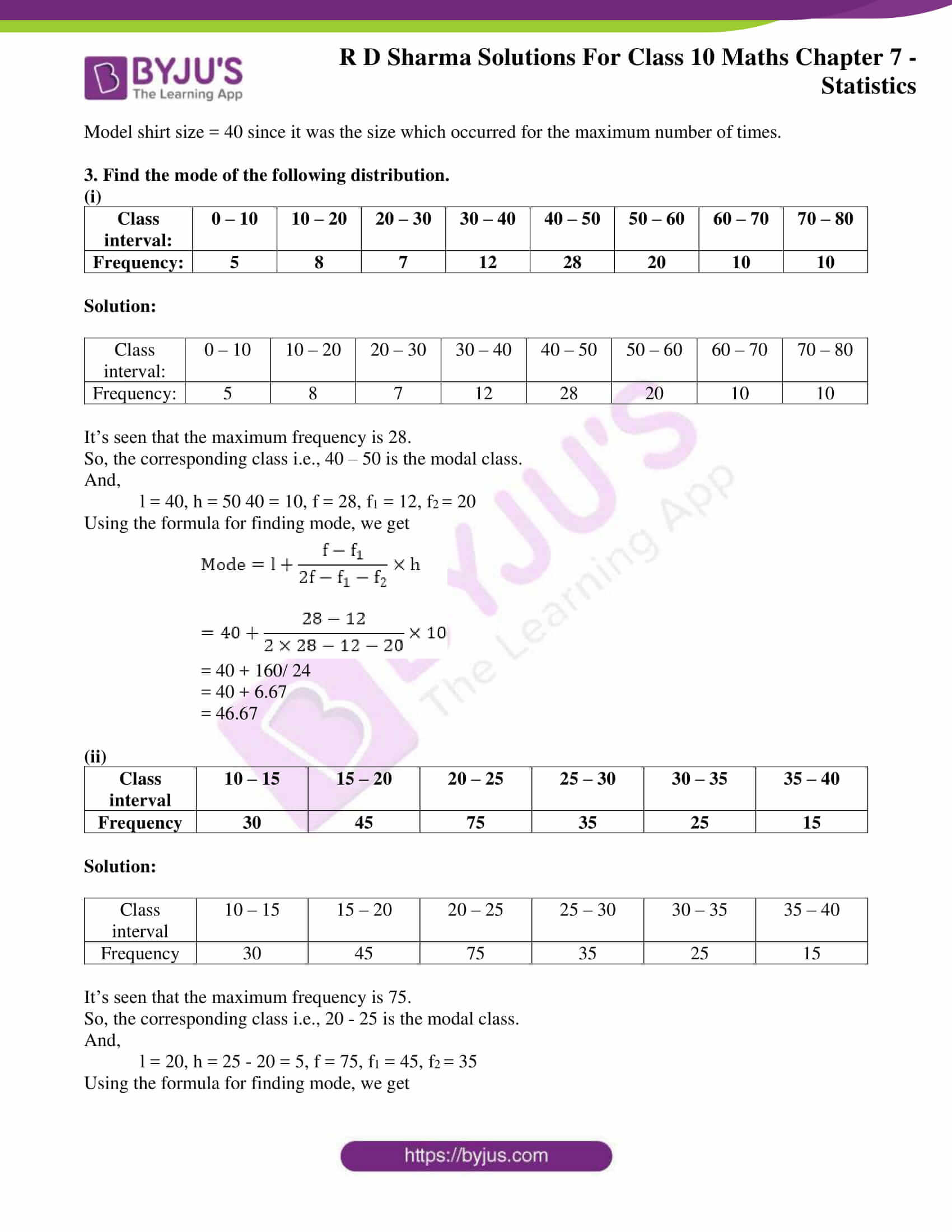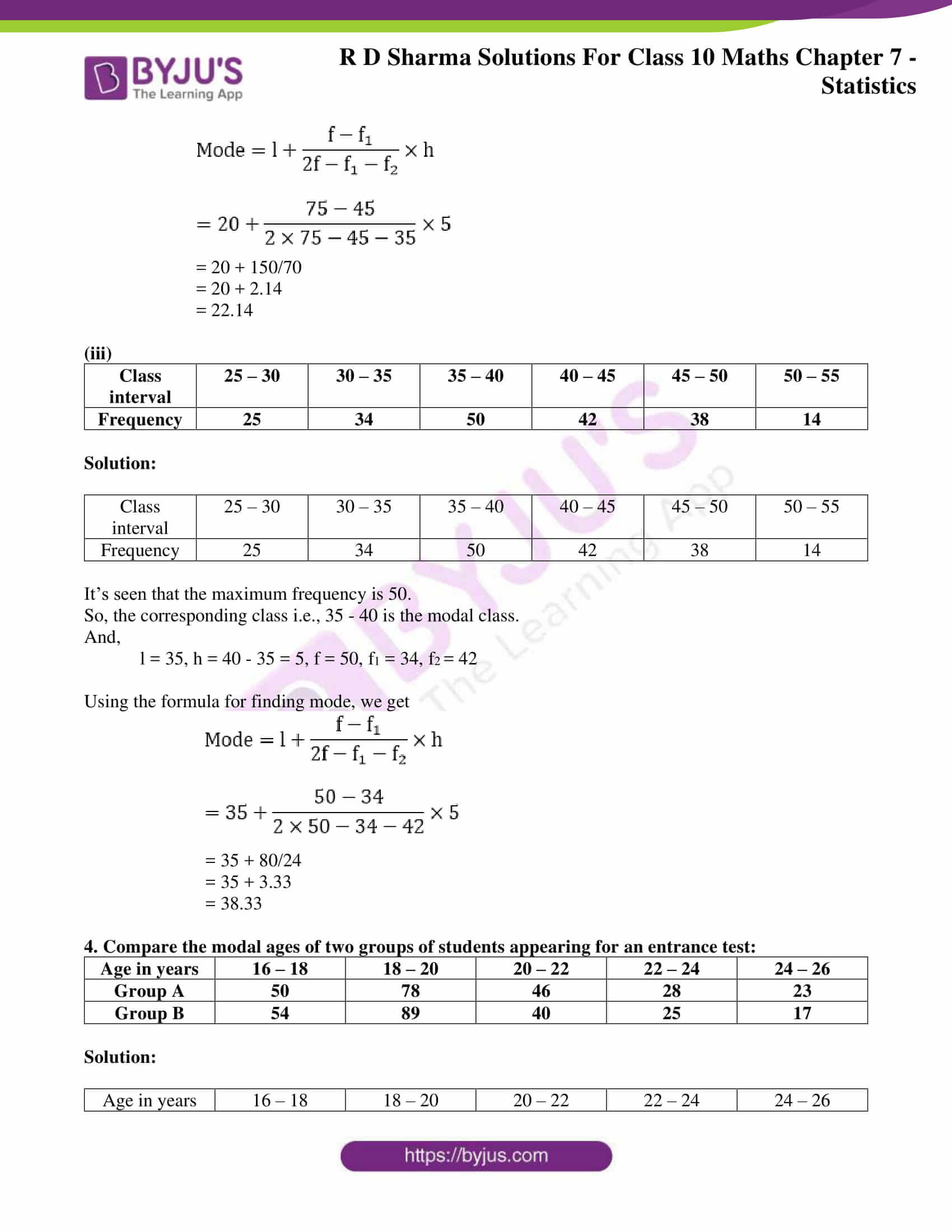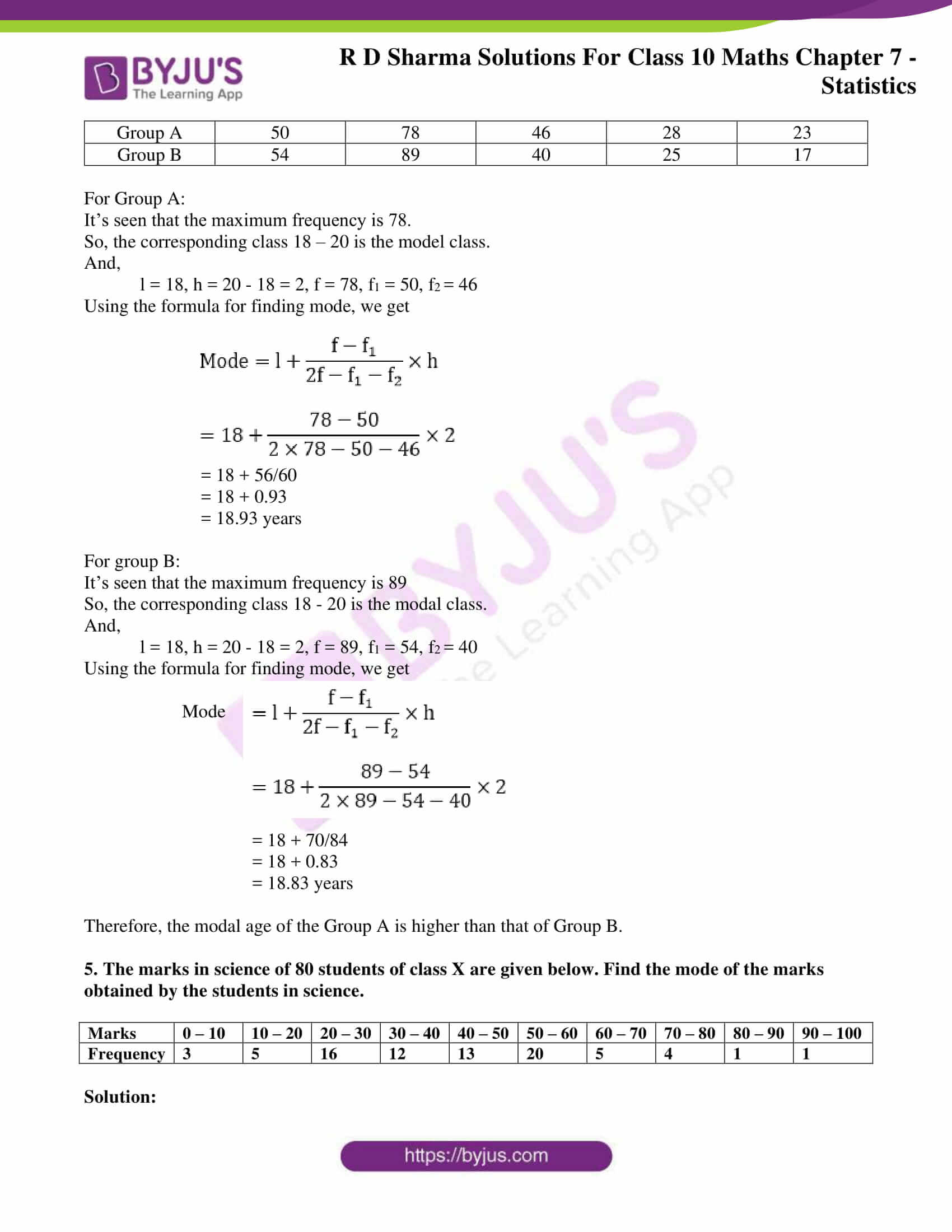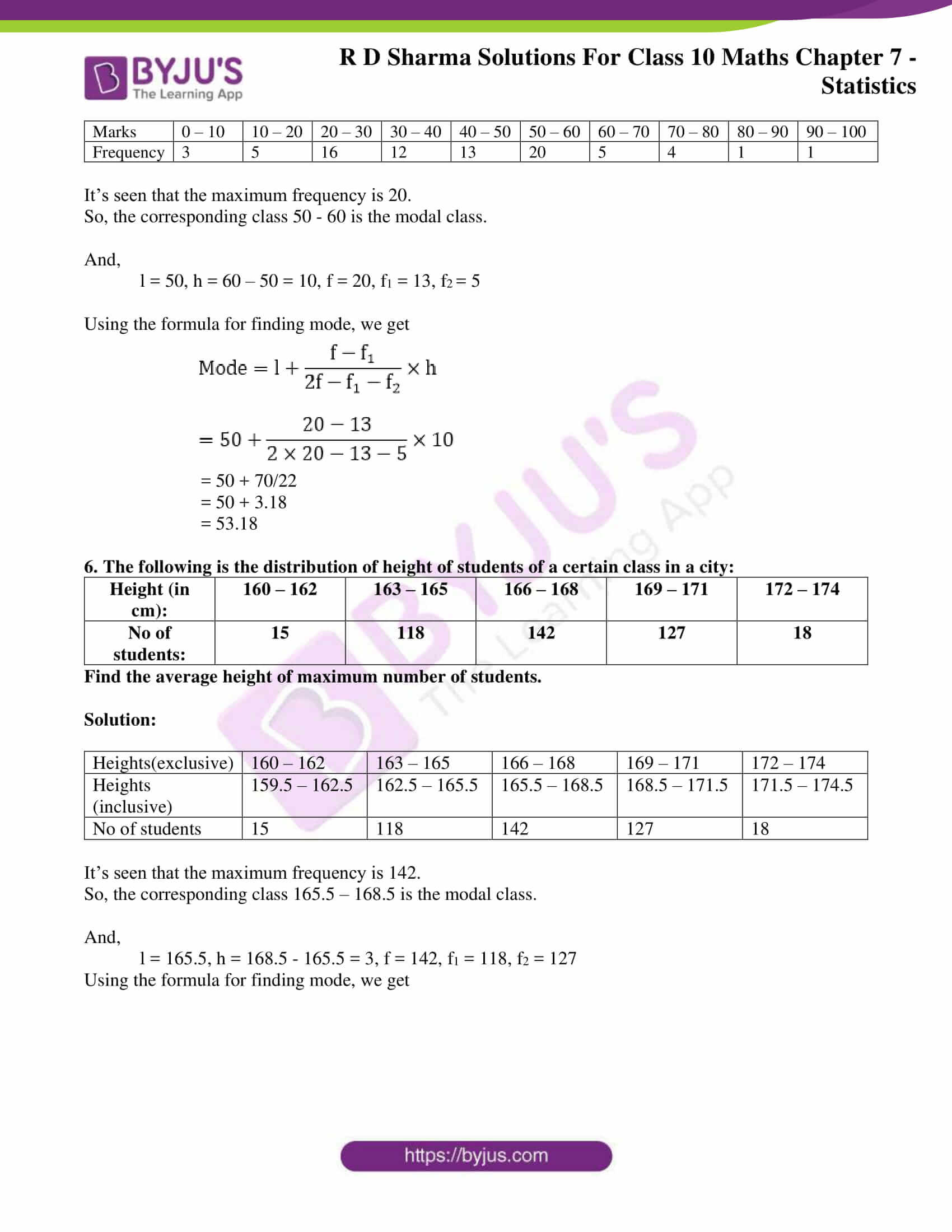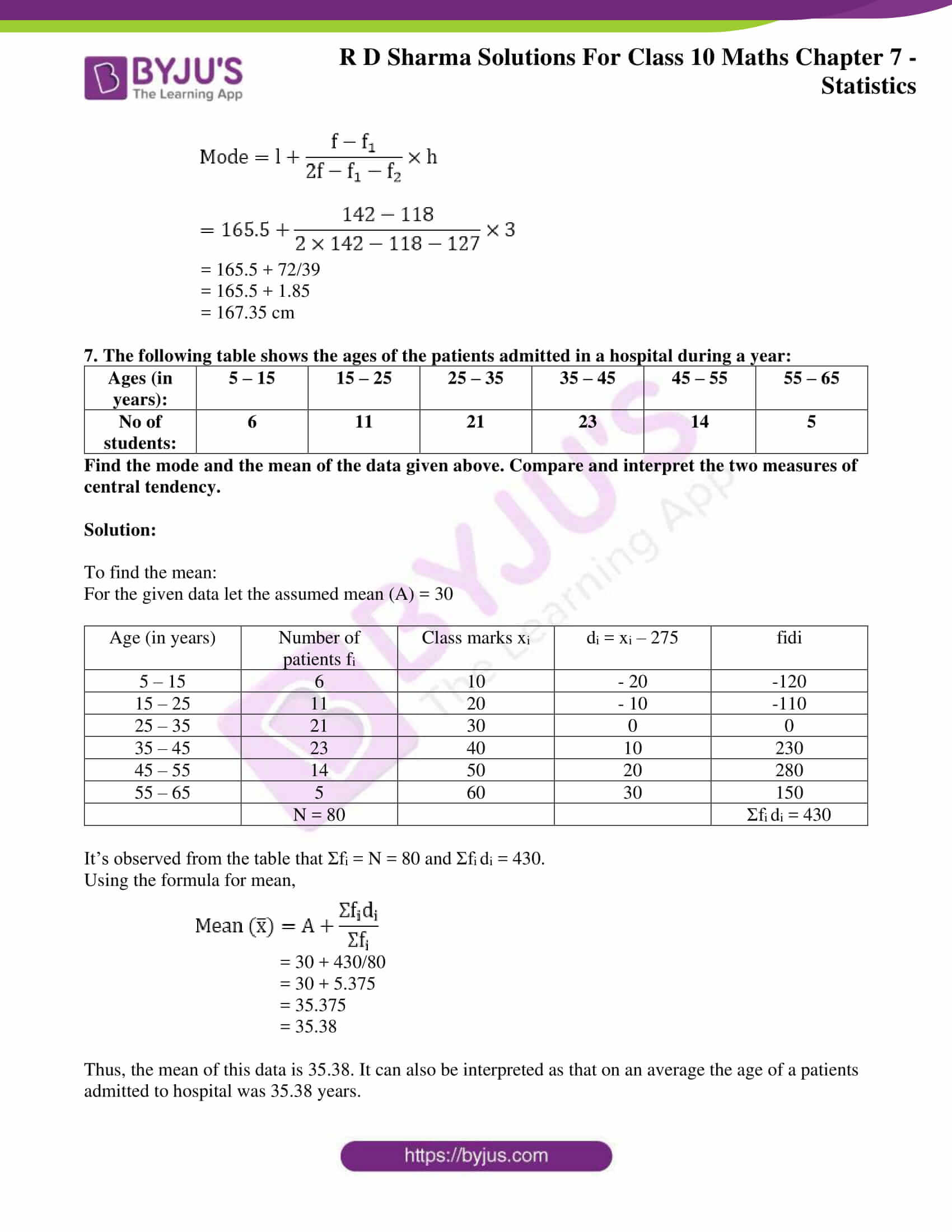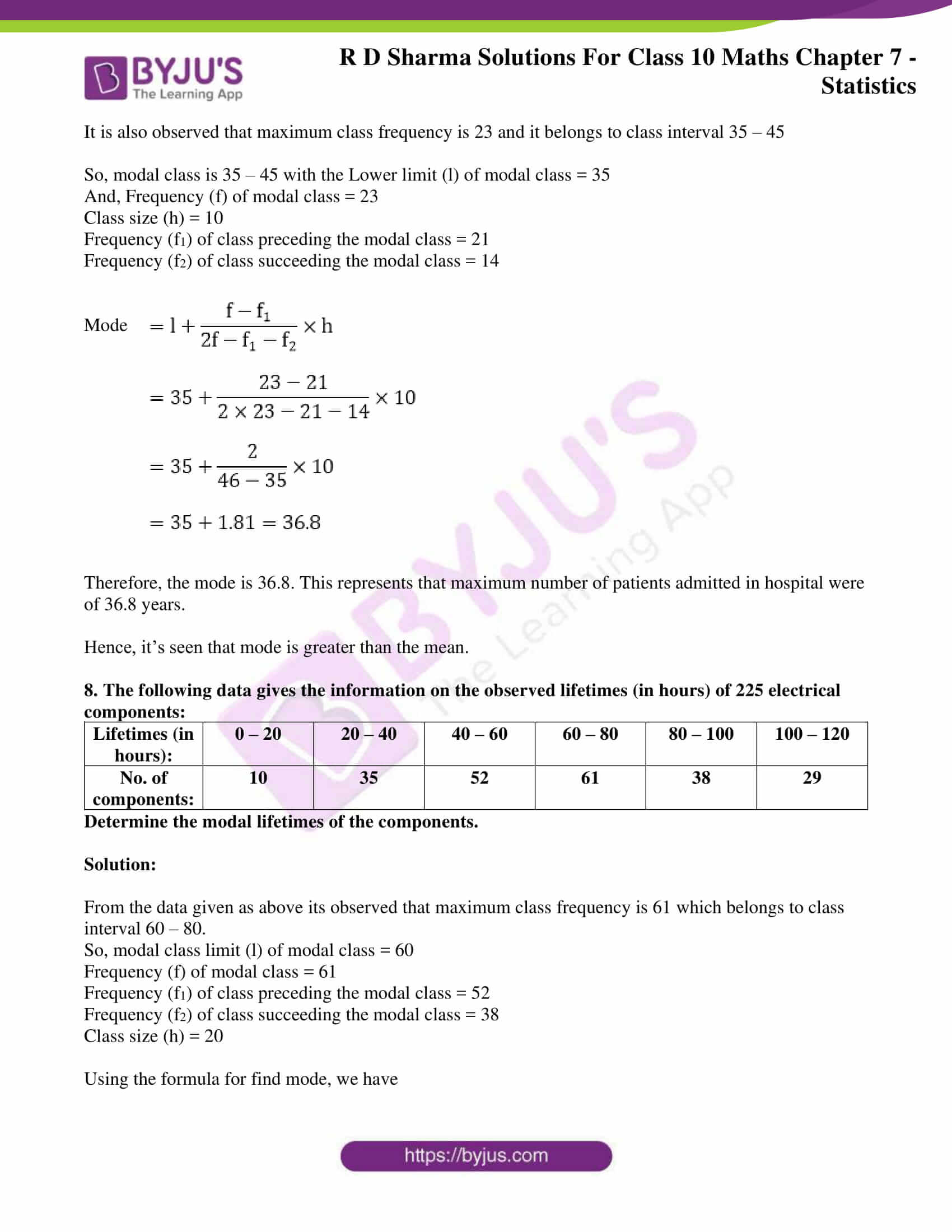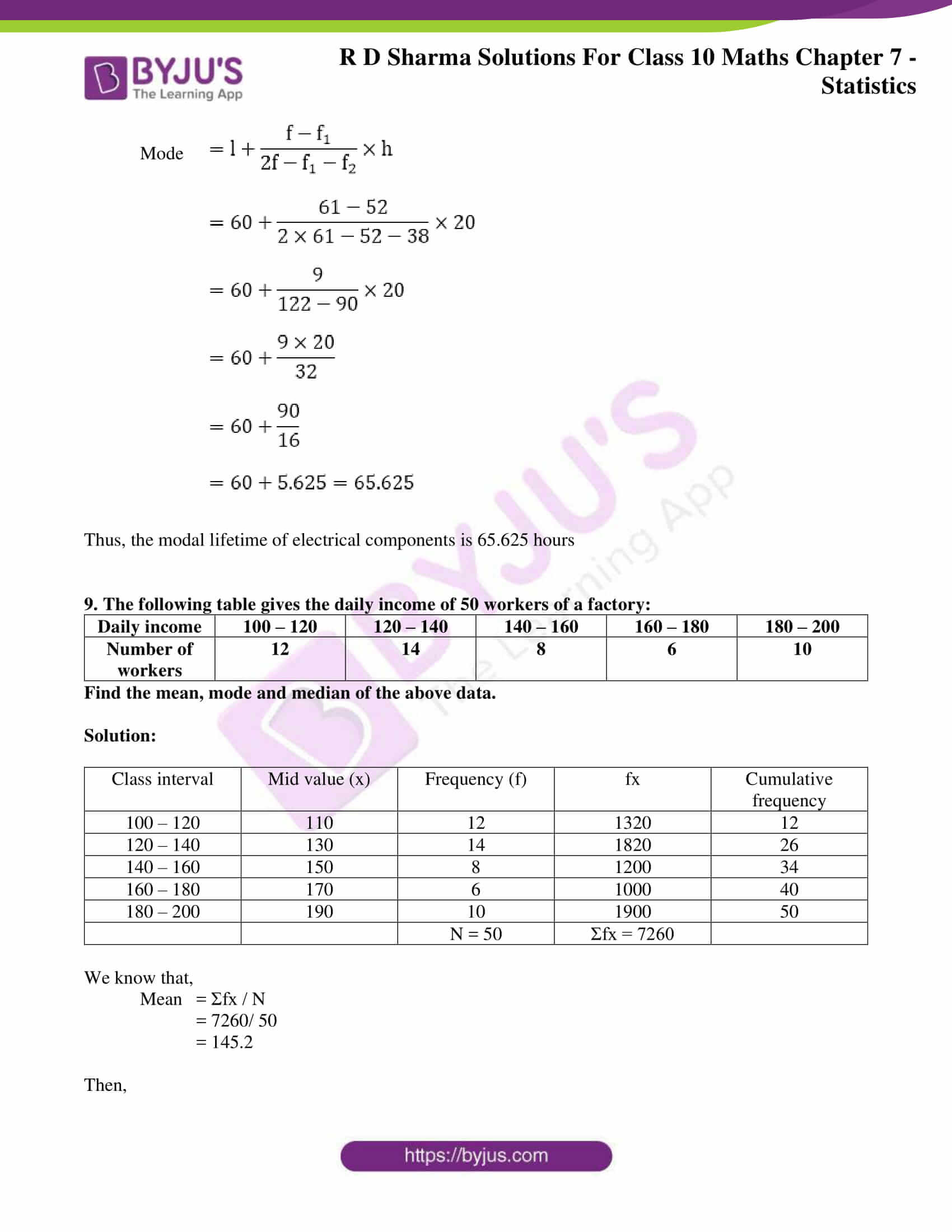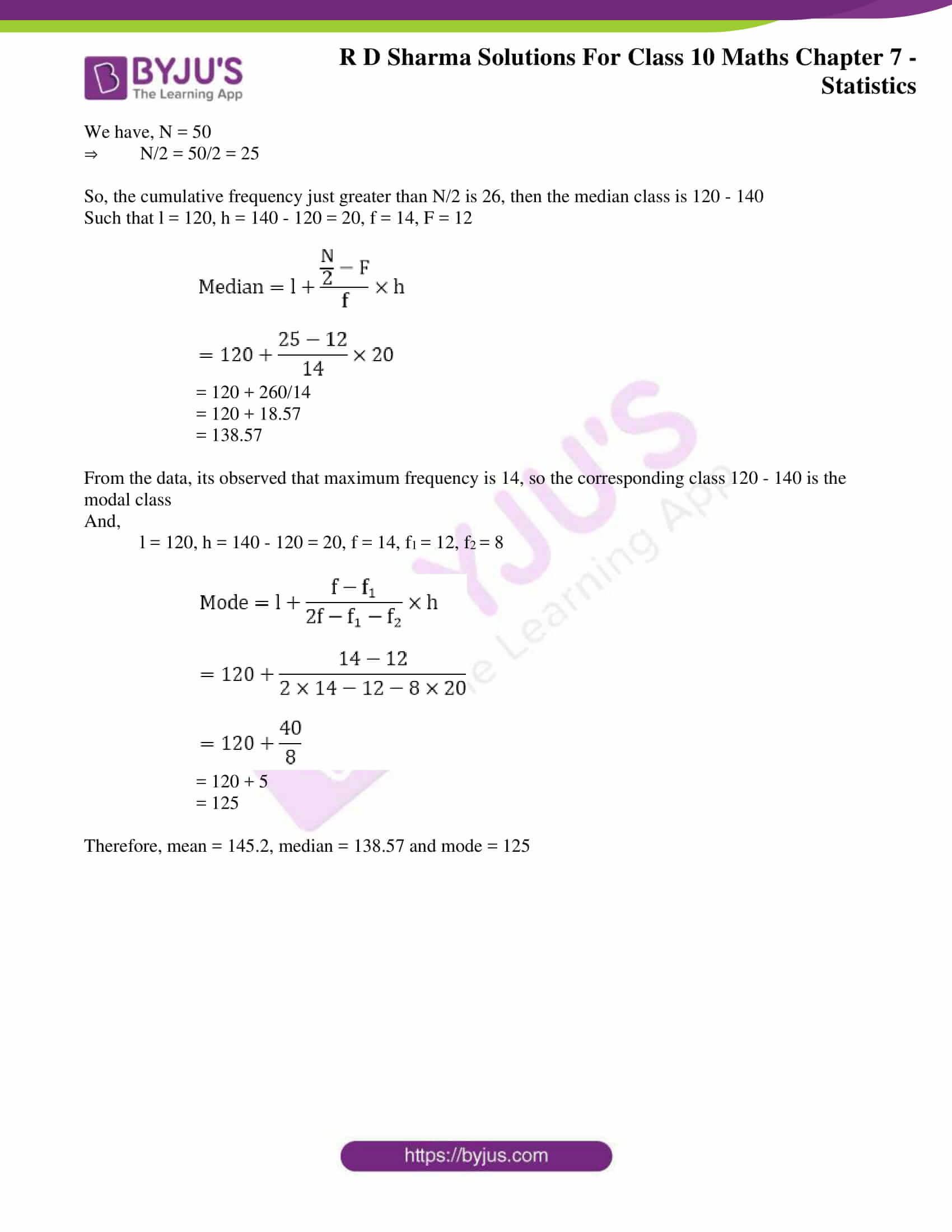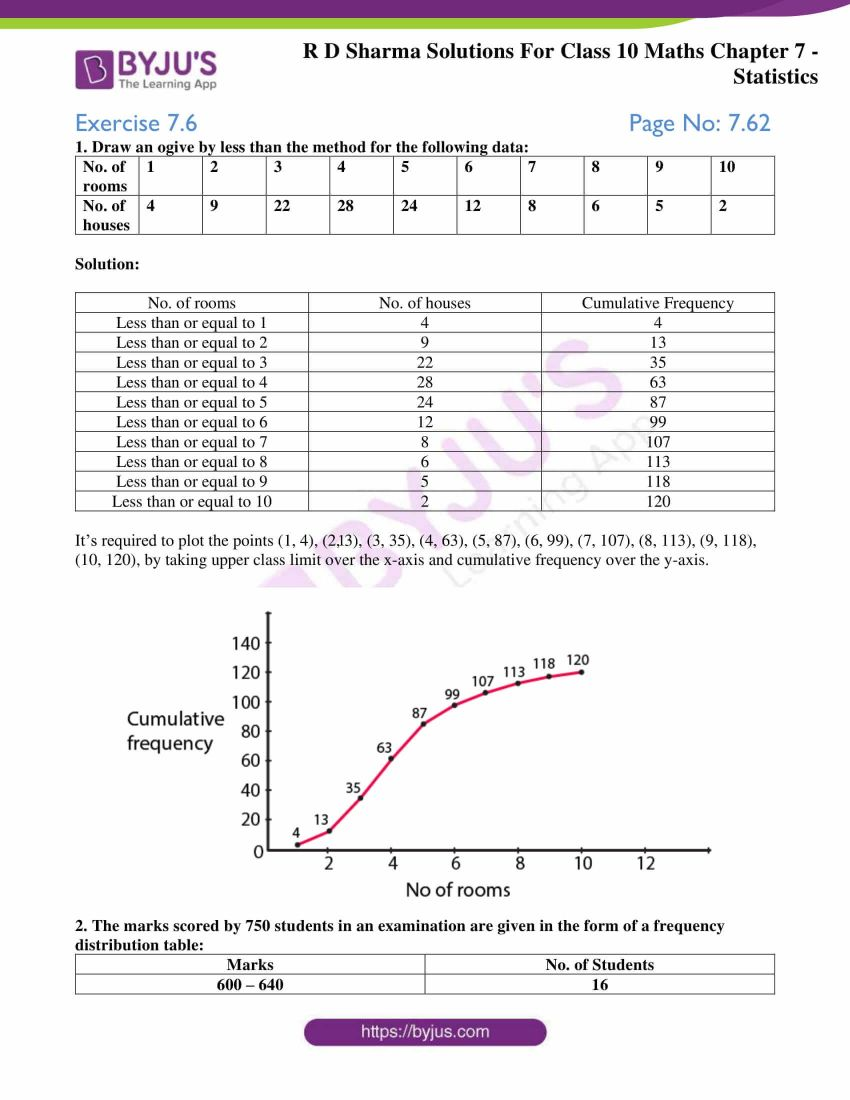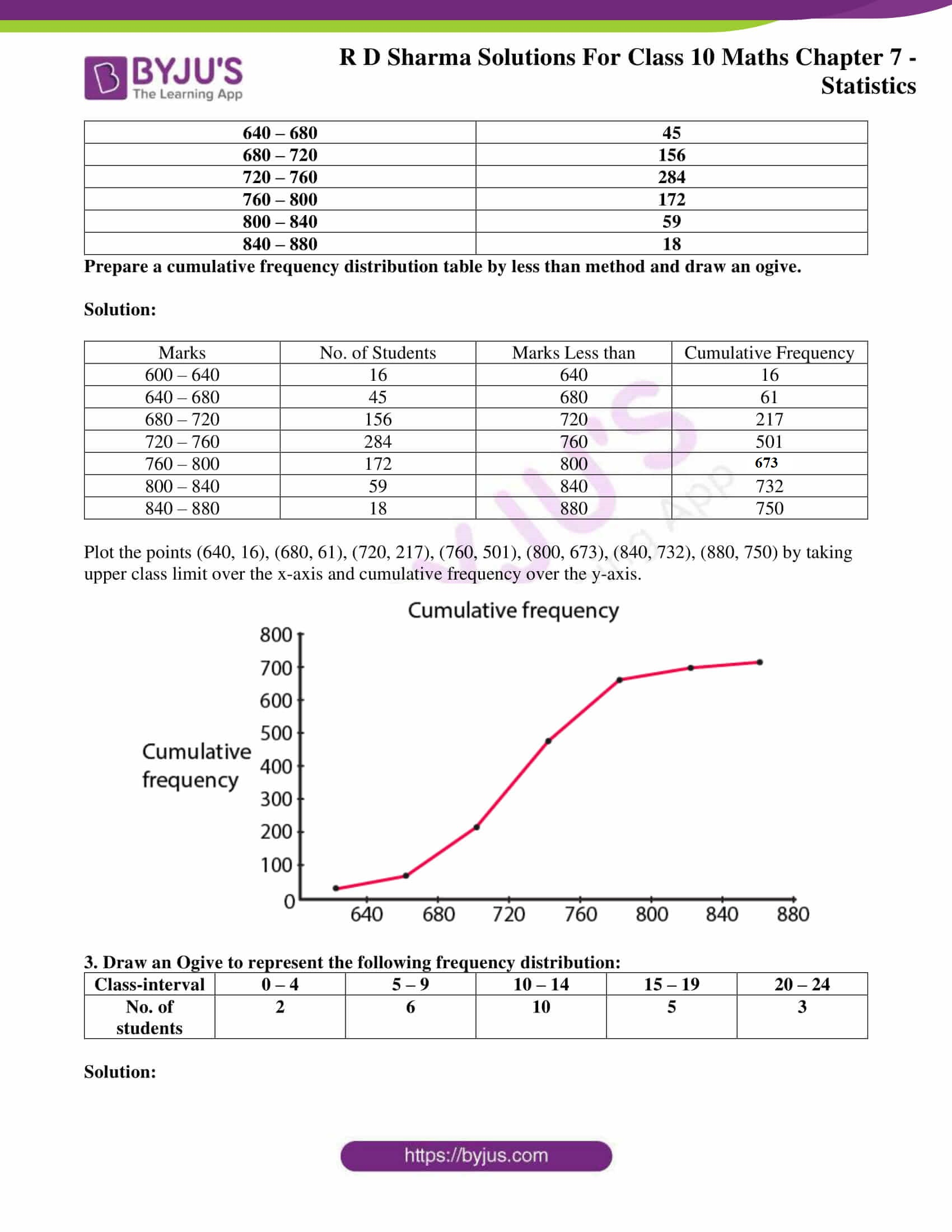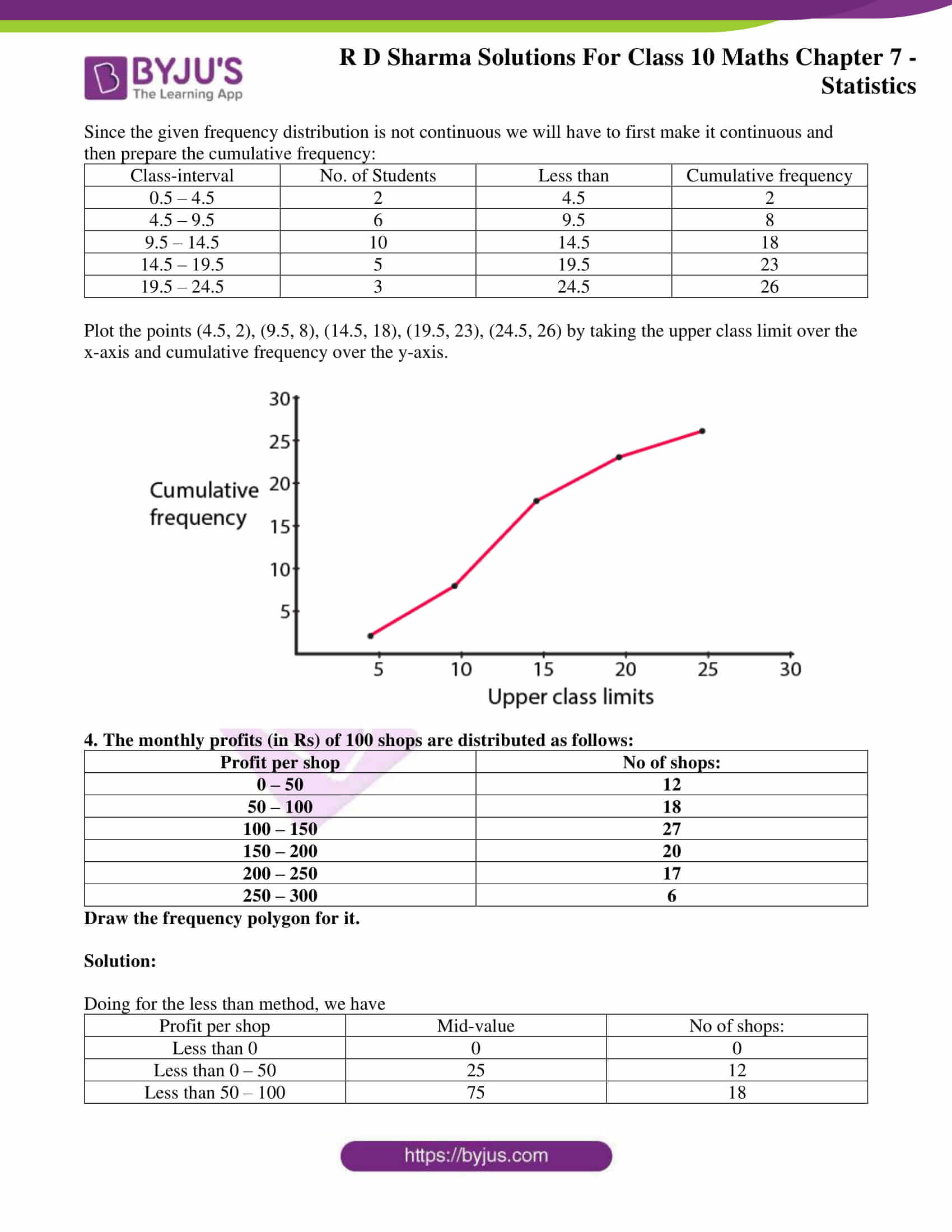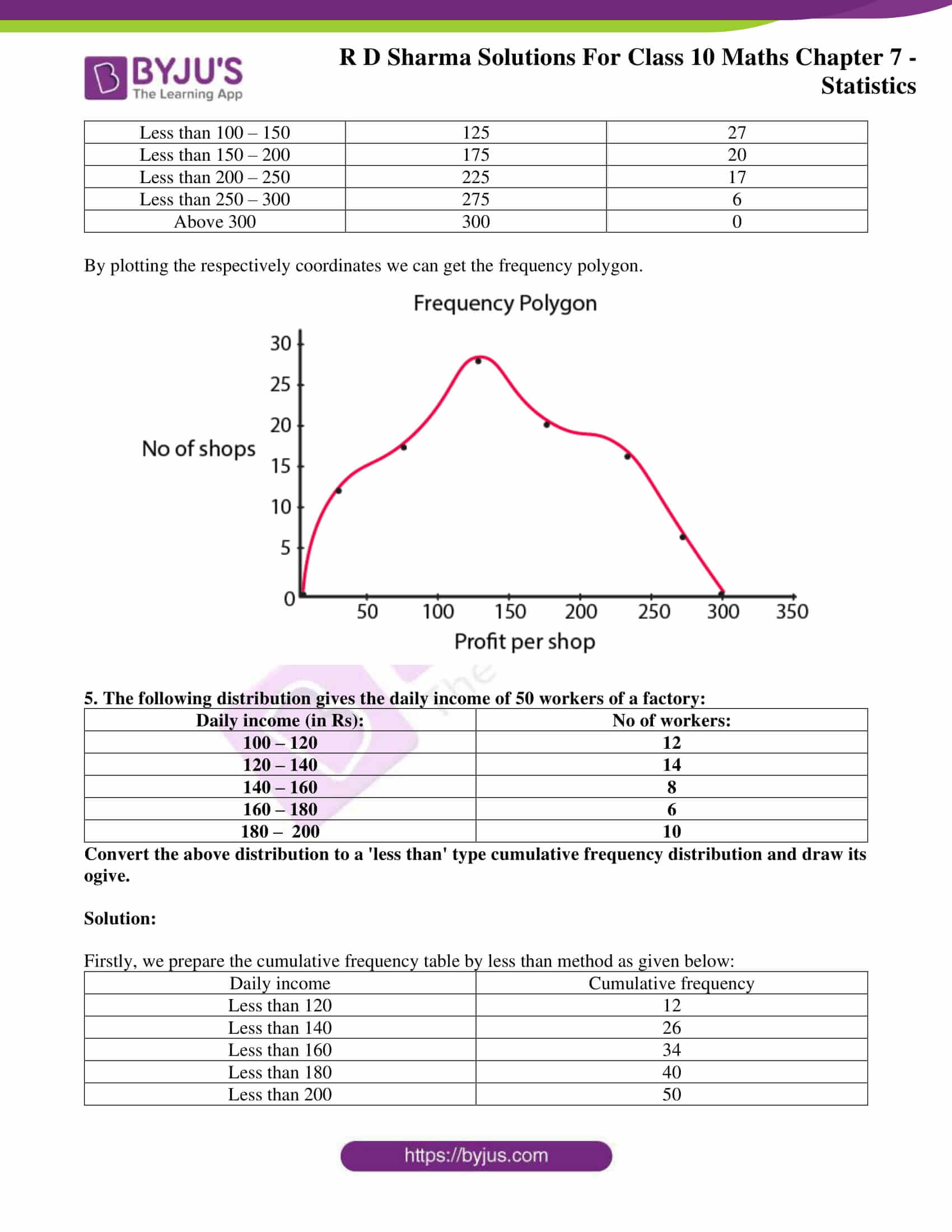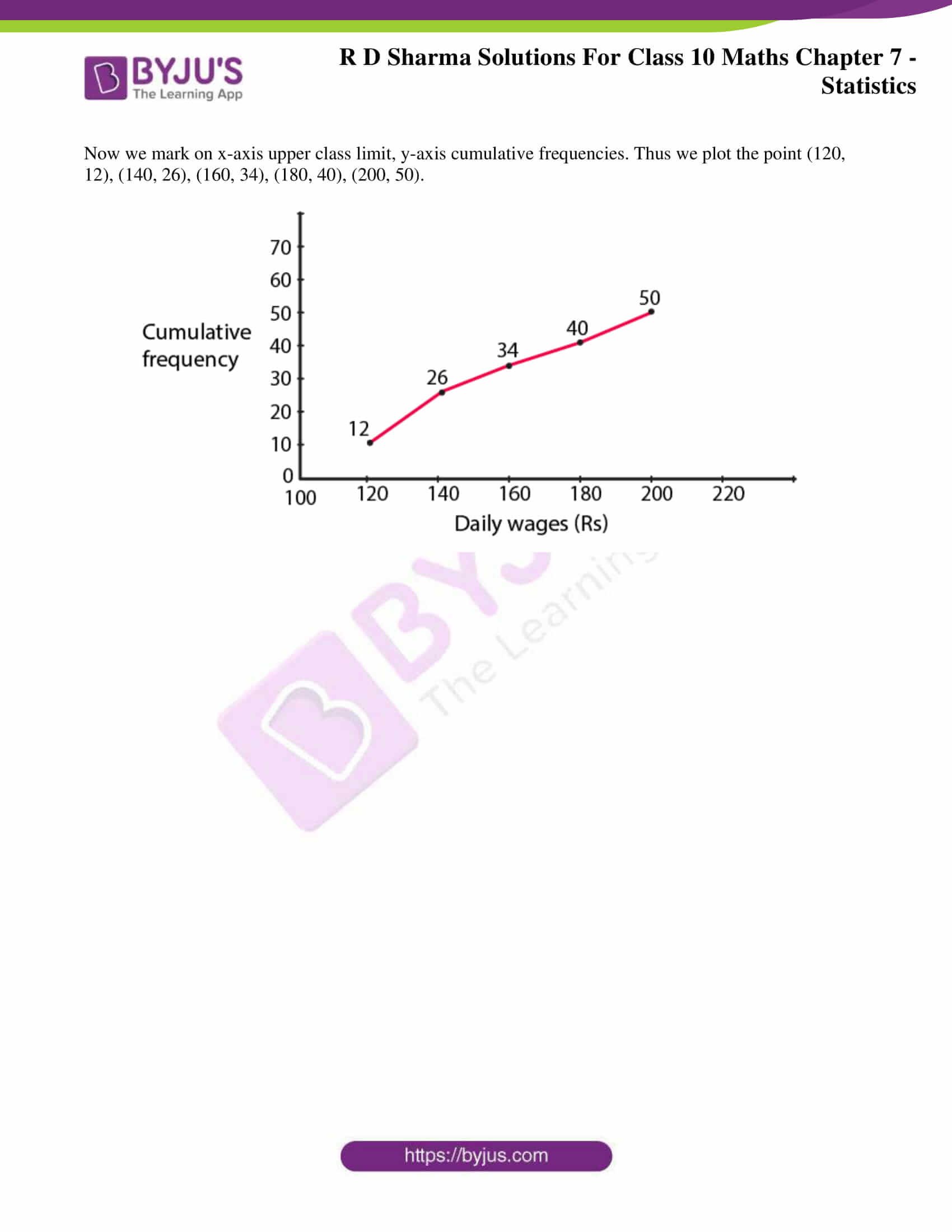### RD Sharma Solutions for Class 10 Maths Chapter 7 Statistics – Exercise-wise

Exercise 7.1 Solutions

Exercise 7.2 Solutions

Exercise 7.3 Solutions

Exercise 7.4 Solutions

Exercise 7.5 Solutions

Exercise 7.6 Solutions

### RD Sharma Solutions for Class 10 Maths Chapter 7 Exercise 7.1 Page No: 7.5

1. Calculate the mean for the following distribution.

 x: 5 6 7 8 9 f: 4 8 14 11 3

Solution:

 x f fx 5 4 20 6 8 48 7 14 98 8 11 88 9 3 27 N = 40 Σ fx = 281

Mean = Σ fx/ N = 281/40

∴ Mean = 7.025

2. Find the mean of the following data.

 x: 19 21 23 25 27 29 31 f: 13 15 16 18 16 15 13

Solution:

 x f fx 19 13 247 21 15 315 23 16 368 25 18 450 27 16 432 29 15 435 31 13 403 N = 106 Σ fx = 2620

Mean = Σ fx/ N = 2620/106

∴ Mean = 25

3. If the mean of the following data is 20.6. Find the value of p.

 x: 10 15 p 25 35 f: 3 10 25 7 5

Solution:

 x f fx 10 3 30 15 10 150 p 25 25p 25 7 175 35 5 175 N = 50 Σ fx = 530 + 25p

We know that,

Mean = Σ fx/ N = (2620 + 25p)/ 50

Given,

Mean = 20.6

⇒ 20.6 = (530 + 25p)/ 50

(20.6 x 50) – 530 = 25p

p = 500/ 25

∴ p = 20

4. If the mean of the following data is 15, find p.

 x: 5 10 15 20 25 f: 6 p 6 10 5

Solution:

 x f fx 5 6 30 10 p 10p 15 6 90 20 10 200 25 5 125 N = p + 27 Σ fx = 445 + 10p

We know that,

Mean = Σ fx/ N = (445 + 10p)/ (p + 27)

Given,

Mean = 15

⇒ 15 = (445 + 10p)/ (p + 27)

15p + 405 = 445 + 10p

5p = 40

∴ p = 8

5. Find the value of p for the following distribution, whose mean is 16.6.

 x: 8 12 15 p 20 25 30 f: 12 16 20 24 16 8 4

Solution:

 x f fx 8 12 96 12 16 192 15 20 300 P 24 24p 20 16 320 25 8 200 30 4 120 N = 100 Σ fx = 1228 + 24p

We know that,

Mean = Σ fx/ N = (1228 + 24p)/ 100

Given,

Mean = 16.6

⇒ 16.6 = (1228 + 24p)/ 100

1660 = 1228 + 24p

24p = 432

∴ p = 18

6. Find the missing value of p for the following distribution, whose mean is 12.58.

 x: 5 8 10 12 p 20 25 f: 2 5 8 22 7 4 2

Solution:

 x f fx 5 2 10 8 5 40 10 8 80 12 22 264 P 7 7p 20 4 80 25 2 50 N = 50 Σ fx = 524 + 7p

We know that,

Mean = Σ fx/ N = (524 + 7p)/ 50

Given,

Mean = 12.58

⇒ 12.58 = (524 + 7p)/ 50

629 = 524 + 7p

7p = 629 – 524 = 105

∴ p = 15

7. Find the missing frequency (p) for the following distribution, whose mean is 7.68.

 x: 3 5 7 9 11 13 f: 6 8 15 p 8 4

Solution:

 x f fx 3 6 18 5 8 40 7 15 105 9 p 9p 11 8 88 13 4 52 N = 41 + p Σ fx = 303 + 9p

We know that,

Mean = Σ fx/ N = (303 + 9p)/ (41 + p)

Given,

Mean = 7.68

⇒ 7.68 = (303 + 9p)/ (41 + p)

7.68(41 + p) = 303 + 9p

7.68p + 314.88 = 303 + 9p

1.32p = 11.88

∴ p = 11.88/1.32 = 9

### RD Sharma Solutions for Class 10 Maths Chapter 7 Exercise 7.2 Page No: 7.5

1. The number of telephone calls received at an exchange per interval for 250 successive one-minute intervals is given in the following frequency table.

 No. of calls (x) 0 1 2 3 4 5 6 No. of intervals (f) 15 24 29 46 54 43 39

Compute the mean number of calls per interval.

Solution:

Let the assumed mean(A) = 3

 No. of calls xi No. of intervals fi ui = xi – A = xi – 3 fi ui 0 15 -3 -45 1 24 -2 -48 2 29 -1 -29 3 46 0 0 4 54 1 54 5 43 2 86 6 39 3 117 N = 250 Σ fixi = 135

Mean number of calls = A + Σ fixi / N

= 3 + 135/250

= (750 + 135)/ 250 = 885/ 250

= 3.54

2. Five coins were simultaneously tossed 1000 times, and at each toss, the number of heads was observed. The number of tosses during which 0, 1, 2, 3, 4 and 5 heads were obtained are shown in the table below. Find the mean number of heads per toss.

 No. of heads per toss (x) 0 1 2 3 4 5 No. of tosses (f) 38 144 342 287 164 25

Solution:

Let the assumed mean(A) = 2

 No. of heads per toss xi No. of intervals fi ui = xi – A = xi – 2 fi ui 0 38 -2 -76 1 144 -1 -144 2 342 0 0 3 287 1 287 4 164 2 328 5 25 3 75 N = 1000 Σ fixi = 470

Mean number of heads per toss = A + Σ fixi / N

= 2 + 470/1000

= 2 + 0.470

= 2.470

3. The following table gives the number of branches and number of plants in the garden of a school.

 No of branches (x) 2 3 4 5 6 No of plants (f) 49 43 57 38 13

Calculate the average number of branches per plant.

Solution:

Let the assumed mean (A) = 4

 No. of branches xi No. of plants fi ui = xi − A = xi − 4 fi ui 2 49 -2 -98 3 43 -1 -43 4 57 0 0 5 38 1 38 6 13 2 26 N = 200 Σ fixi = -77

Average number of branches per plant = A + Σ fixi / N = 4 + (-77/200)

= 4 -77/200

= (800 -77)/200

= 3.615

4. The following table gives the number of children of 150 families in a village.

 No of children (x) 0 1 2 3 4 5 No of families (f) 10 21 55 42 15 7

Find the average number of children per family.

Solution:

Let the assumed mean (A) = 2

 No. of children xi No. of families fi ui = xi − A = xi − 2 fi ui 0 10 -2 -20 1 21 -1 -21 2 55 0 0 3 42 1 42 4 15 2 30 5 7 3 21 N = 150 Σ fixi = 52

Average number of children for family = A + Σ fixi / N = 2 + 52/150

= (300 +52)/150

= 352/150

= 2.35 (corrected to neat decimal)

### RD Sharma Solutions for Class 10 Maths Chapter 7 Exercise 7.3 Page No: 7.22

1. The following table gives the distribution of total household expenditure (in rupees) of manual workers in a city.

 Expenditure (in rupees) (x) Frequency (fi) Expenditure (in rupees) (xi) Frequency (fi) 100 – 150 24 300 – 350 30 150 – 200 40 350 – 400 22 200 – 250 33 400 – 450 16 250 – 300 28 450 – 500 7

Find the average expenditure (in rupees) per household.

Solution:

Let the assumed mean (A) = 275

 Class interval Mid value (xi) di = xi – 275 ui = (xi – 275)/50 Frequency fi fiui 100 – 150 125 -150 -3 24 -72 150 – 200 175 -100 -2 40 -80 200 – 250 225 -50 -1 33 -33 250 – 300 275 0 0 28 0 300 – 350 325 50 1 30 30 350 – 400 375 100 2 22 44 400 – 450 425 150 3 16 48 450 – 500 475 200 4 7 28 N = 200 Σ fiui = -35

It’s seen that A = 275 and h = 50

So,

Mean = A + h x (Σfi ui/N)

= 275 + 50 (-35/200)

= 275 – 8.75

= 266.25

2. A survey was conducted by a group of students as a part of their environmental awareness program, in which they collected the following data regarding the number of plants in 200 houses in a locality. Find the mean number of plants per house.

 Number of plants 0 – 2 2 – 4 4 – 6 6 – 8 8 – 10 10 – 12 12 – 14 Number of houses 1 2 1 5 6 2 3

Which method did you use for finding the mean, and why?

Solution:

From the given data,

To find the class interval, we know that,

Classmarks (xi) = (upper class limit + lower class limit)/2

Now, let’s compute xi and fixi by the following:

 Number of plants Number of houses (fi) xi fixi 0 – 2 1 1 1 2 – 4 2 3 6 4 – 6 1 5 5 6 – 8 5 7 35 8 – 10 6 9 54 10 – 12 2 11 22 12 – 14 3 13 39 Total N = 20 Σ fiui = 162

Here,

Mean = Σ fiui / N

= 162/ 20

= 8.1

Thus, the mean number of plants in a house is 8.1.

We have used the direct method, as the values of class mark xi and fi are very small.

3. Consider the following distribution of daily wages of workers of a factory.

 Daily wages (in ₹) 100 – 120 120 – 140 140 – 160 160 – 180 180 – 200 Number of workers 12 14 8 6 10

Find the mean daily wages of the workers of the factory by using an appropriate method.

Solution:

Let the assumed mean (A) = 150

 Class interval Mid value xi di = xi – 150 ui = (xi – 150)/20 Frequency fi fiui 100 – 120 110 -40 -2 12 -24 120 – 140 130 -20 -1 14 -14 140 – 160 150 0 0 8 0 160 – 180 170 20 1 6 6 180 – 200 190 40 2 10 20 N= 50 Σ fiui = -12

It’s seen that,

A = 150 and h = 20

So,

Mean = A + h x (Σfi ui/N)

= 150 + 20 x (-12/50)

= 150 – 24/5

= 150 = 4.8

= 145.20

4. Thirty women were examined in a hospital by a doctor, and the number of heartbeats per minute recorded and summarised is as follows. Find the mean heartbeats per minute for these women, choosing a suitable method.

 Number of heartbeats per minute 65 – 68 68 – 71 71 – 74 74 – 77 77 – 80 80 – 83 83 – 86 Number of women 2 4 3 8 7 4 2

Solution:

Using the relation (xi) = (Upper class limit + Lower class limit)/ 2

And class size of this data = 3

Let the assumed mean (A) = 75.5

So, let’s calculate di, ui, fiui as follows:

 Number of heartbeats per minute Number of women (fi) xi di = xi – 75.5 ui = (xi – 755)/h fiui 65 – 68 2 66.5 -9 -3 -6 68 – 71 4 69.5 -6 -2 -8 71 – 74 3 72.5 -3 -1 -3 74 – 77 8 75.5 0 0 0 77 – 80 7 78.5 3 1 7 80 – 83 4 81.5 6 2 8 83 – 86 2 84.5 9 3 6 N = 30 Σ fiui = 4

From the table, it’s seen that

N = 30 and h = 3

So, the mean = A + h x (Σfi ui/N)

= 75.5 + 3 x (4/30

= 75.5 + 2/5

= 75.9

Therefore, the mean heartbeats per minute for those women are 75.9 beats per minute.

Find the mean of each of the following frequency distributions: (5 – 14)

5.

 Class interval: 0 – 6 6 – 12 12 – 18 18 – 24 24 – 30 Frequency: 6 8 10 9 7

Solution:

Let’s consider the assumed mean (A) = 15

 Class interval Mid-value xi di = xi – 15 ui = (xi – 15)/6 fi fiui 0 – 6 3 -12 -2 6 -12 6 – 12 9 -6 -1 8 -8 12 – 18 15 0 0 10 0 18 – 24 21 6 1 9 9 24 – 30 27 12 2 7 14 N = 40 Σ fiui = 3

From the table, it’s seen that

A = 15 and h = 6

Mean = A + h x (Σfi ui/N)

= 15 + 6 x (3/40)

= 15 + 0.45

= 15.45

6.

 Class interval: 50 – 70 70 – 90 90 – 110 110 – 130 130 – 150 150 – 170 Frequency: 18 12 13 27 8 22

Solution:

Let’s consider the assumed mean (A) = 100

 Class interval Mid-value xi di = xi – 100 ui = (xi – 100)/20 fi fiui 50 – 70 60 -40 -2 18 -36 70 – 90 80 -20 -1 12 -12 90 – 110 100 0 0 13 0 110 – 130 120 20 1 27 27 130 – 150 140 40 2 8 16 150 – 170 160 60 3 22 66 N= 100 Σ fiui = 61

From the table, it’s seen that

A = 100 and h = 20

Mean = A + h x (Σfi ui/N)

= 100 + 20 x (61/100)

= 100 + 12.2

= 112.2

7.

 Class interval 0 – 8 8 – 16 16 – 24 24 – 32 32 – 40 Frequency 6 7 10 8 9

Solution:

Let’s consider the assumed mean (A) = 20

 Class interval Mid-value xi di = xi – 20 ui = (xi – 20)/8 fi fiui 0 – 8 4 -16 -2 6 -12 8 – 16 12 -8 -1 7 -7 16 – 24 20 0 0 10 0 24 – 32 28 8 1 8 8 32 – 40 36 16 2 9 18 N = 40 Σ fiui = 7

From the table, it’s seen that

A = 20 and h = 8

Mean = A + h x (Σfi ui/N)

= 20 + 8 x (7/40)

= 20 + 1.4

= 21.4

8.

 Class intervaL 0 – 6 6 – 12 12 – 18 18 – 24 24 – 30 Frequency 7 5 10 12 6

Solution:

Let’s consider the assumed mean (A) = 15

 Class interval Mid-value xi di = xi – 15 ui = (xi – 15)/6 fi fiui 0 – 6 3 -12 -2 7 -14 6 – 12 9 -6 -1 5 -5 12 – 18 15 0 0 10 0 18 – 24 21 6 1 12 12 24 – 30 27 12 2 6 12 N = 40 Σ fiui = 5

From the table, it’s seen that

A = 15 and h = 6

Mean = A + h x (Σfi ui/N)

= 15 + 6 x (5/40)

= 15 + 0.75

= 15.75

9.

 Class interval 0 – 10 10 – 20 20 – 30 30 – 40 40 – 50 Frequency 9 12 15 10 14

Solution:

Let’s consider the assumed mean (A) = 25

 Class interval Mid-value xi di = xi – 25 ui = (xi – 25)/10 fi fiui 0 – 10 5 -20 -2 9 -18 10 – 20 15 -10 -1 12 -12 20 – 30 25 0 0 15 0 30 – 40 35 10 1 10 10 40 – 50 45 20 2 14 28 N = 60 Σ fiui = 8

From the table, it’s seen that

A = 25 and h = 10

Mean = A + h x (Σfi ui/N)

= 25 + 10 x (8/60)

= 25 + 4/3

= 79/3 = 26.333

10.

 Class interval 0 – 8 8 – 16 16 – 24 24 – 32 32 – 40 Frequency 5 9 10 8 8

Solution:

Let’s consider the assumed mean (A) = 20

 Class interval Mid-value xi di = xi – 20 ui = (xi – 20)/8 fi fiui 0 – 8 4 -16 -2 5 -10 8 – 16 12 -4 -1 9 -9 16 – 24 20 0 0 10 0 24 – 32 28 4 1 8 8 32 – 40 36 16 2 8 16 N = 40 Σ fiui = 5

From the table, it’s seen that

A = 20 and h = 8

Mean = A + h x (Σfi ui/N)

= 20 + 8 x (5/40)

= 20 + 1

= 21

11.

 Class interval 0 – 8 8 – 16 16 – 24 24 – 32 32 – 40 Frequency 5 6 4 3 2

Solution:

Let’s consider the assumed mean (A) = 20

 Class interval Mid-value xi di = xi – 20 ui = (xi – 20)/8 fi fiui 0 – 8 4 -16 -2 5 -12 8 – 16 12 -8 -1 6 -8 16 – 24 20 0 0 4 0 24 – 32 28 8 1 3 9 32 – 40 36 16 2 2 14 N = 20 Σ fiui = -9

From the table, it’s seen that

A = 20 and h = 8

Mean = A + h x (Σfi ui/N)

= 20 + 6 x (-9/20)

= 20 – 72/20

= 20 – 3.6

= 16.4

12.

 Class interval 10 – 30 30 – 50 50 – 70 70 – 90 90 – 110 110 – 130 Frequency 5 8 12 20 3 2

Solution:

Let’s consider the assumed mean (A) = 60

 Class interval Mid-value xi di = xi –60 ui = (xi – 60)/20 fi fiui 10 – 30 20 -40 -2 5 -10 30 – 50 40 -20 -1 8 -8 50 – 70 60 0 0 12 0 70 – 90 80 20 1 20 20 90 – 110 100 40 2 3 6 110 – 130 120 60 3 2 6 N = 50 Σ fiui = 14

From the table, it’s seen that

A = 60 and h = 20

Mean = A + h x (Σfi ui/N)

= 60 + 20 x (14/50)

= 60 + 28/5

= 60 + 5.6

= 65.6

13.

 Class interval 25 – 35 35 – 45 45 – 55 55 – 65 65 – 75 Frequency 6 10 8 12 4

Solution:

Let’s consider the assumed mean (A) = 50

 Class interval Mid-value xi di = xi – 50 ui = (xi – 50)/10 fi fiui 25 – 35 30 -20 -2 6 -12 35 – 45 40 -10 -1 10 -10 45 – 55 50 0 0 8 0 55 – 65 60 10 1 12 12 65 – 75 70 20 2 4 8 N = 40 Σ fiui = -2

From the table, it’s seen that

A = 50 and h = 10

Mean = A + h x (Σfi ui/N)

= 50 + 10 x (-2/40)

= 50 – 0.5

= 49.5

14.

 Class interval 25 – 29 30 – 34 35 – 39 40 – 44 45 – 49 50 – 54 55 – 59 Frequency 14 22 16 6 5 3 4

Solution:

Let’s consider the assumed mean (A) = 42

 Class interval Mid-value xi di = xi – 42 ui = (xi – 42)/5 fi fiui 25 – 29 27 -15 -3 14 -42 30 – 34 32 -10 -2 22 -44 35 – 39 37 -5 -1 16 -16 40 – 44 42 0 0 6 0 45 – 49 47 5 1 5 5 50 – 54 52 10 2 3 6 55 – 59 57 15 3 4 12 N = 70 Σ fiui = -79

From the table, it’s seen that

A = 42 and h = 5

Mean = A + h x (Σfi ui/N)

= 42 + 5 x (-79/70)

= 42 – 79/14

= 42 – 5.643

= 36.357

### RD Sharma Solutions for Class 10 Maths Chapter 7 Exercise 7.4 Page No: 7.34

1. Following are the lives in hours of 15 pieces of the components of an aircraft engine. Find the median.

715, 724, 725, 710, 729, 745, 694, 699, 696, 712, 734, 728, 716, 705, 719.

Solution:

Arranging the given data in ascending order, we have

694, 696, 699, 705, 710, 712, 715, 716, 719, 721, 725, 728, 729, 734, 745

As the number of terms is an old number, i.e., N = 15

We use the following procedure to find the median.

Median = (N + 1)/2 th term

= (15 + 1)/2 th term

= 8th term

So, the 8th term in the arranged order of the given data should be the median.

Therefore, 716 is the median of the data.

2. The following is the distribution of the height of students of a certain class in a certain city:

 Height (in cm) 160 – 162 163 – 165 166 – 168 169 – 171 172 – 174 No. of students 15 118 142 127 18

Find the median height.

Solution:

 Class interval (exclusive) Class interval  (inclusive) Class interval frequency Cumulative frequency 160 – 162 159.5 – 162.5 15 15 163 – 165 162.5 – 165.5 118 133(F) 166 – 168 165.5 – 168.5 142(f) 275 169 – 171 168.5 – 171.5 127 402 172 – 174 171.5 – 174.5 18 420 N = 420

Here, we have N = 420,

So, N/2 = 420/ 2 = 210

The cumulative frequency just greater than N/2 is 275, then 165.5 – 168.5 is the median class, such that

L = 165.5, f = 142, F = 133 and h = (168.5 – 165.5) = 3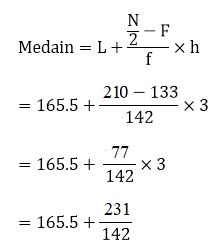= 165.5 + 1.63

= 167.13

3. Following is the distribution of I.Q. of 100 students. Find the median I.Q.

 I.Q: 55 – 64 65 – 74 75 – 84 85 – 94 95 – 104 105 – 114 115 – 124 125 – 134 135 – 144 No of students: 1 2 9 22 33 22 8 2 1

Solution:

 Class interval (exclusive) Class interval  (inclusive) Class interval frequency Cumulative frequency 55 – 64 54.5 – 64-5 1 1 65 – 74 64.5 – 74.5 2 3 75 – 84 74.5 – 84.5 9 12 85 – 94 84.5 – 94.5 22 34(F) 95 – 104 94.5 – 104.5 33(f) 67 105 – 114 104.5 – 114.5 22 89 115 – 124 114.5 – 124.5 8 97 125 – 134 124.5 – 134.5 2 98 135 – 144 134.5 – 144.5 1 100 N = 100

Here, we have N = 100,

So, N/2 = 100/ 2 = 50

The cumulative frequency just greater than N/ 2 is 67, then the median class is (94.5 – 104.5), such that L = 94.5, F = 33, h = (104.5 – 94.5) = 10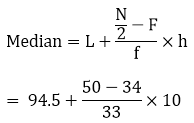= 94.5 + 4.85

= 99.35

4. Calculate the median from the following data:

 Rent (in Rs): 15 – 25 25 – 35 35 – 45 45 – 55 55 – 65 65 – 75 75 – 85 85 – 95 No of houses: 8 10 15 25 40 20 15 7

Solution:

 Class interval Frequency Cumulative frequency 15 – 25 8 8 25 – 35 10 18 35 – 45 15 33 45 – 55 25 58(F) 55 – 65 40(f) 98 65 – 75 20 118 75 – 85 15 133 85 – 95 7 140 N = 140

Here, we have N = 140,

So, N/2 = 140/ 2 = 70

The cumulative frequency just greater than N/ 2 is 98, then median class is 55 – 65, such that L = 55, f = 40, F = 58, h = 65 – 55 = 10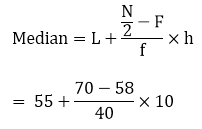= 55 + 3 = 58

5. Calculate the median from the following data:

 Marks below: 10 – 20 20 – 30 30 – 40 40 – 50 50 – 60 60 – 70 70 – 80 85 – 95 No of students: 15 35 60 84 96 127 198 250

Solution:

 Marks below No. of students Class interval Frequency Cumulative frequency 10 15 0 – 10 15 15 20 35 10 – 20 20 35 30 60 20 – 30 25 60 40 84 30 – 40 24 84 50 96 40 – 50 12 96(F) 60 127 50 – 60 31(f) 127 70 198 60 – 70 71 198 80 250 70 – 80 52 250 N = 250

Here, we have N = 250,

So, N/2 = 250/ 2 = 125

The cumulative frequency just greater than N/ 2 is 127, then median class is 50 – 60, such that L = 50, f = 31, F = 96, h = 60 -50 = 10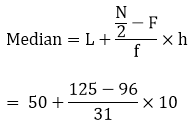= 50 + 9.35

= 59.35

6. Calculate the missing frequency from the following distribution, it being given that the median of the distribution is 24.

 Age in years: 0 – 10 10 – 20 20 – 30 30 – 40 40 – 50 No of persons: 5 25 ? 18 7

Solution:

Let the unknown frequency be taken as x,

 Class interval Frequency Cumulative frequency 0 – 10 5 5 10 – 20 25 30(F) 20 – 30 x (f) 30 + x 30 – 40 18 48 + x 40 – 50 7 55 + x N = 170

It’s given that

Median = 24

Then, median class = 20 – 30; L = 20, h = 30 -20 = 10, f = x, F = 30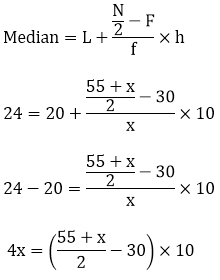4x = 275 + 5x – 300

4x – 5x = – 25

– x = – 25

x = 25

Therefore, the Missing frequency = 25

7. The following table gives the frequency distribution of married women by age at marriage.

 Age (in years) Frequency Age (in years) Frequency 15 – 19 53 40 – 44 9 20 – 24 140 45 – 49 5 25 – 29 98 45 – 49 3 30 – 34 32 55 – 59 3 35 – 39 12 60 and above 2

Calculate the median and interpret the results.

Solution:

 Class interval (exclusive) Class interval (inclusive) Frequency Cumulative frequency 15 – 19 14.5 – 19.5 53 53 (F) 20 – 24 19.5 – 24.5 140 (f) 193 25 – 29 24.5 – 29.5 98 291 30 – 34 29.5 – 34.5 32 323 35 – 39 34.5 – 39.5 12 335 40 – 44 39.5 – 44.5 9 344 45 – 49 44.5 – 49.5 5 349 50 – 54 49.5 – 54.5 3 352 55 – 54 54.5 – 59.5 3 355 60 and above 59.5 and above 2 357 N =357

Here, we have N = 357,

So, N/2 = 357/ 2 = 178.5

The cumulative frequency just greater than N/2 is 193, so then the median class is (19.5 – 24.5), such that l = 19.5, f = 140, F = 53, h = 25.5 – 19.5 = 5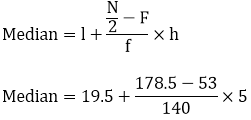Median = 23.98

This means nearly half the women were married between the ages of 15 and 25.

8. The following table gives the distribution of the lifetime of 400 neon lamps:

 Lifetime (in hours) Number of lamps 1500 – 2000 14 2000 – 2500 56 2500 – 3000 60 3000 – 3500 86 3500 – 4000 74 4000 – 4500 62 4500 – 5000 48

Find the median life.

Solution:

 Lifetime Number of lamps fi Cumulative frequency (cf) 1500 – 2000 14 14 2000 – 2500 56 70 2500 – 3000 60 130(F) 3000 – 3500 86(f) 216 3500 – 4000 74 290 4000 – 4500 62 352 4500 – 5000 48 400 N = 400

It’s seen that the cumulative frequency just greater than n/2 (400/2 = 200) is 216, and it belongs to the class interval 3000 – 3500, which becomes the Median class = 3000 – 3500

Lower limits (l) of median class = 3000 and,

Frequency (f) of median class = 86

Cumulative frequency (cf) of class preceding median class = 130

And the Class size (h) = 500

Thus, by calculating the median by the formula, we get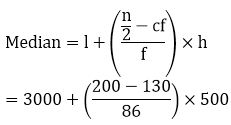= 3000 + (35000/86)

= 3406.98

Thus, the median lifetime of lamps is 3406.98 hours.

9. The distribution below gives the weight of 30 students in a class. Find the median weight of students.

 Weight (in kg): 40 – 45 45 – 50 50 – 55 55 – 60 60 – 65 65 – 70 70 – 75 No of students: 2 3 8 6 6 3 2

Solution:

 Weight (in kg) Number of students fi Cumulative frequency (cf) 40 – 45 2 2 45 – 50 3 5 50 – 55 8 13 55 – 60 6 19 60 – 65 6 25 65 – 70 3 28 70 – 75 2 30

It’s seen that the cumulative frequency just greater than n/ 2 (i.e. 30/ 2 = 15) is 19, belongs to class interval 55 – 60.

So, it’s chosen that

Median class = 55 – 60

Lower limit (l) of median class = 55

Frequency (f) of median class = 6

Cumulative frequency (cf) = 13

And, Class size (h) = 5

Thus, by calculating the median by the formula, we get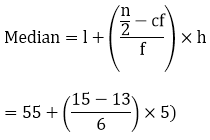= 55 + 10/6 = 56.666

So, the median weight is 56.67 kg.

10. Find the missing frequencies and the median for the following distribution if the mean is 1.46

 No. of accidents 0 1 2 3 4 5 Total Frequencies (no. of days) 46 ? ? 25 10 5 200

Solution:

 No. of accidents (x) No. of days (f) fx 0 46 0 1 x x 2 y 2y 3 25 75 4 10 40 5 5 25 N = 200 Sum = x + 2y + 140

It’s given that N = 200

⇒ 46 + x + y + 25 + 10 + 5 = 200

⇒ x + y = 200 – 46 – 25 – 10 – 5

⇒ x + y = 114 —- (i)

And also given, Mean = 1.46

⇒ Sum/ N = 1.46

⇒ (x + 2y + 140)/ 200 = 1.46

⇒ x + 2y = 292 – 140

⇒ x + 2y = 152 —- (ii)

Subtract equation (i) from equation (ii), and we get

x + 2y – x – y = 152 – 114

⇒ y = 38

Now, on putting the value of y in equation (i), we find x = 114 – 38 = 76

Thus, the table becomes

 No. of accidents (x) No. of days (f) Cumulative frequency 0 46 46 1 76 122 2 38 160 3 25 185 4 10 195 5 5 200 N = 200

It’s seen that,

N = 200 N/2 = 200/2 = 100

So, the cumulative frequency is more than N/2 is 122

Therefore, the median is 1.

### RD Sharma Solutions for Class 10 Maths Chapter 7 Exercise 7.5 Page No: 7.34

1. Find the mode of the following data:

(i) 3, 5, 7, 4, 5, 3, 5, 6, 8, 9, 5, 3, 5, 3, 6, 9, 7, 4

(ii) 3, 3, 7, 4, 5, 3, 5, 6, 8, 9, 5, 3, 5, 3, 6, 9, 7, 4

(iii) 15, 8, 26, 25, 24, 15, 18, 20, 24, 15, 19, 15

Solution:

(i)

 Value (x) 3 4 5 6 7 8 9 Frequency (f) 4 2 5 2 2 1 2

Thus, the mode = 5 since it occurs the maximum number of times.

(ii)

 Value (x) 3 4 5 6 7 8 9 Frequency (f) 5 2 4 2 2 1 2

Thus, the mode = 3 since it occurs the maximum number of times.

(iii)

 Value (x) 8 15 18 19 20 24 25 Frequency (f) 1 4 1 1 1 2 1

Thus, the mode = 15 since it occurs the maximum number of times.

2. The shirt size worn by a group of 200 persons, who bought the shirt from a store, are as follows:

 Shirt size 37 38 39 40 41 42 43 44 Number of persons 15 25 39 41 36 17 15 12

Find the model shirt size worn by the group.

Solution:

 Shirt size 37 38 39 40 41 42 43 44 Number of persons 15 25 39 41 36 17 15 12

From the data, it’s observed that

Model shirt size = 40 since it was the size which occurred for the maximum number of times.

3. Find the mode of the following distribution.

(i)

 Class interval 0 – 10 10 – 20 20 – 30 30 – 40 40 – 50 50 – 60 60 – 70 70 – 80 Frequency 5 8 7 12 28 20 10 10

Solution:

 Class interval 0 – 10 10 – 20 20 – 30 30 – 40 40 – 50 50 – 60 60 – 70 70 – 80 Frequency 5 8 7 12 28 20 10 10

It’s seen that the maximum frequency is 28.

So, the corresponding class, i.e., 40 – 50 is the modal class.

And,

l = 40, h = 50 40 = 10, f = 28, f1 = 12, f= 20

Using the formula for finding mode, we get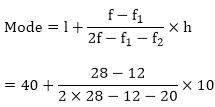= 40 + 160/ 24

= 40 + 6.67

= 46.67

(ii)

 Class interval 10 – 15 15 – 20 20 – 25 25 – 30 30 – 35 35 – 40 Frequency 30 45 75 35 25 15

Solution:

 Class interval 10 – 15 15 – 20 20 – 25 25 – 30 30 – 35 35 – 40 Frequency 30 45 75 35 25 15

It’s seen that the maximum frequency is 75.

So, the corresponding class, i.e., 20 – 25 is the modal class.

And,

l = 20, h = 25 – 20 = 5, f = 75, f1 = 45, f= 35

Using the formula for finding mode, we get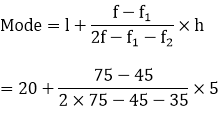= 20 + 150/70

= 20 + 2.14

= 22.14

(iii)

 Class interval 25 – 30 30 – 35 35 – 40 40 – 45 45 – 50 50 – 55 Frequency 25 34 50 42 38 14

Solution:

 Class interval 25 – 30 30 – 35 35 – 40 40 – 45 45 – 50 50 – 55 Frequency 25 34 50 42 38 14

It’s seen that the maximum frequency is 50.

So, the corresponding class, i.e., 35 – 40 is the modal class.

And,

l = 35, h = 40 – 35 = 5, f = 50, f1 = 34, f= 42

Using the formula for finding mode, we get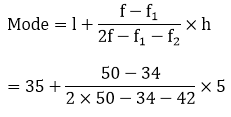= 35 + 80/24

= 35 + 3.33

= 38.33

4. Compare the modal ages of two groups of students appearing for an entrance test.

 Age in years 16 – 18 18 – 20 20 – 22 22 – 24 24 – 26 Group A 50 78 46 28 23 Group B 54 89 40 25 17

Solution:

 Age in years 16 – 18 18 – 20 20 – 22 22 – 24 24 – 26 Group A 50 78 46 28 23 Group B 54 89 40 25 17

For Group A:

It’s seen that the maximum frequency is 78.

So, the corresponding class 18 – 20 is the model class.

And,

l = 18, h = 20 – 18 = 2, f = 78, f1 = 50, f= 46

Using the formula for finding mode, we get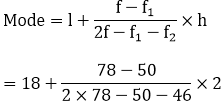= 18 + 56/60

= 18 + 0.93

= 18.93 years

For group B:

It’s seen that the maximum frequency is 89.

So, the corresponding class 18 – 20 is the modal class.

And,

l = 18, h = 20 – 18 = 2, f = 89, f1 = 54, f= 40

Using the formula for finding mode, we get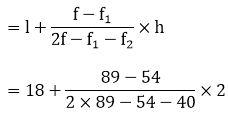Mode

= 18 + 70/84

= 18 + 0.83

= 18.83 years

Therefore, the modal age of Group A is higher than that of Group B.

5. The marks in science for 80 students of Class X are given below. Find the mode of the marks obtained by the students in science.

 Marks 0 – 10 10 – 20 20 – 30 30 – 40 40 – 50 50 – 60 60 – 70 70 – 80 80 – 90 90 – 100 Frequency 3 5 16 12 13 20 5 4 1 1

Solution:

 Marks 0 – 10 10 – 20 20 – 30 30 – 40 40 – 50 50 – 60 60 – 70 70 – 80 80 – 90 90 – 100 Frequency 3 5 16 12 13 20 5 4 1 1

It’s seen that the maximum frequency is 20.

So, the corresponding class 50 – 60 is the modal class.

And,

l = 50, h = 60 – 50 = 10, f = 20, f1 = 13, f= 5

Using the formula for finding mode, we get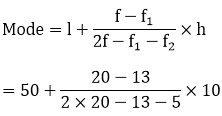= 50 + 70/22

= 50 + 3.18

= 53.18

6. The following is the distribution of height of students of a certain class in a city:

 Height (in cm): 160 – 162 163 – 165 166 – 168 169 – 171 172 – 174 No of students: 15 118 142 127 18

Find the average height of the maximum number of students.

Solution:

 Heights (exclusive) 160 – 162 163 – 165 166 – 168 169 – 171 172 – 174 Heights (inclusive) 159.5 – 162.5 162.5 – 165.5 165.5 – 168.5 168.5 – 171.5 171.5 – 174.5 No. of students 15 118 142 127 18

It’s seen that the maximum frequency is 142.

So, the corresponding class 165.5 – 168.5 is the modal class.

And,

l = 165.5, h = 168.5 – 165.5 = 3, f = 142, f1 = 118, f2 = 127

Using the formula for finding mode, we get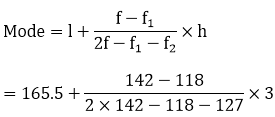= 165.5 + 72/39

= 165.5 + 1.85

= 167.35 cm

7. The following table shows the ages of the patients admitted to a hospital during a year:

 Ages (in years) 5 – 15 15 – 25 25 – 35 35 – 45 45 – 55 55 – 65 No of students 6 11 21 23 14 5

Find the mode and the mean of the data given above. Compare and interpret the two measures of central tendency.

Solution:

To find the mean:

For the given data, let the assumed mean (A) = 30

 Age (in years) Number of patients fi Classmarks xi di = xi – 275 fidi 5 – 15 6 10 – 20 -120 15 – 25 11 20 – 10 -110 25 – 35 21 30 0 0 35 – 45 23 40 10 230 45 – 55 14 50 20 280 55 – 65 5 60 30 150 N = 80 Σfi di = 430

It’s observed from the table that Σfi = N = 80 and Σfdi = 430.

Using the formula for mean,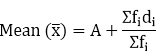= 30 + 430/80

= 30 + 5.375

= 35.375

= 35.38

Thus, the mean of this data is 35.38. It can also be interpreted as that, on an average, the age of patients admitted to the hospital was 35.38 years.

It is also observed that the maximum class frequency is 23, and it belongs to the class interval 35 – 45

So, the modal class is 35 – 45 with the Lower limit (l) of modal class = 35

And, Frequency (f) of modal class = 23

Class size (h) = 10

Frequency (f1) of class preceding the modal class = 21

Frequency (f2) of class succeeding the modal class = 14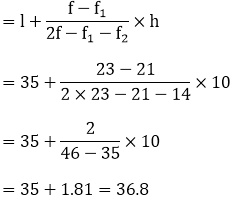Mode

Therefore, the mode is 36.8. This represents that the maximum number of patients admitted to the hospital was of 36.8 years.

Hence, it’s seen that mode is greater than the mean.

8. The following data gives information on the observed lifetimes (in hours) of 225 electrical components:

 Lifetimes (in hours) 0 – 20 20 – 40 40 – 60 60 – 80 80 – 100 100 – 120 No. of components 10 35 52 61 38 29

Determine the modal lifetimes of the components.

Solution:

From the data given above, it’s observed that the maximum class frequency is 61, which belongs to the class interval 60 – 80.

So, modal class limit (l) of modal class = 60

Frequency (f) of modal class = 61

Frequency (f1) of class preceding the modal class = 52

Frequency (f2) of class succeeding the modal class = 38

Class size (h) = 20

Using the formula for find mode, we have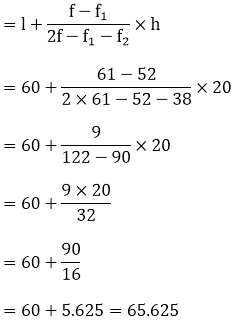Mode

Thus, the modal lifetime of electrical components is 65.625 hours.

9. The following table gives the daily income of 50 workers of a factory:

 Daily income 100 – 120 120 – 140 140 – 160 160 – 180 180 – 200 Number of workers 12 14 8 6 10

Find the mean, mode and median of the above data.

Solution:

 Class interval Mid value (x) Frequency (f) fx Cumulative frequency 100 – 120 110 12 1320 12 120 – 140 130 14 1820 26 140 – 160 150 8 1200 34 160 – 180 170 6 1000 40 180 – 200 190 10 1900 50 N = 50 Σfx = 7260

We know that,

Mean = Σfx / N

= 7260/ 50

= 145.2

Then,

We have, N = 50

⇒ N/2 = 50/2 = 25

So, the cumulative frequency just greater than N/2 is 26, then the median class is 120 – 140.

Such that l = 120, h = 140 – 120 = 20, f = 14, F = 12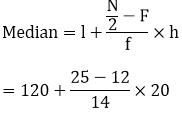= 120 + 260/14

= 120 + 18.57

= 138.57

From the data, it’s observed that the maximum frequency is 14, so the corresponding class 120 – 140 is the modal class.

And,

l = 120, h = 140 – 120 = 20, f = 14, f= 12, f= 8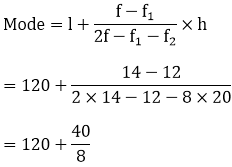= 120 + 5

= 125

Therefore, mean = 145.2, median = 138.57 and mode = 125

### RD Sharma Solutions for Class 10 Maths Chapter 7 Exercise 7.6 Page No: 7.62

1. Draw an ogive by less than the method for the following data.

 No. of rooms 1 2 3 4 5 6 7 8 9 10 No. of houses 4 9 22 28 24 12 8 6 5 2

Solution:

 No. of rooms No. of houses Cumulative Frequency Less than or equal to 1 4 4 Less than or equal to 2 9 13 Less than or equal to 3 22 35 Less than or equal to 4 28 63 Less than or equal to 5 24 87 Less than or equal to 6 12 99 Less than or equal to 7 8 107 Less than or equal to 8 6 113 Less than or equal to 9 5 118 Less than or equal to 10 2 120

It’s required to plot the points (1, 4), (2, 13), (3, 35), (4, 63), (5, 87), (6, 99), (7, 107), (8, 113), (9, 118), (10, 120), by taking upper-class limit over the x-axis and cumulative frequency over the y-axis.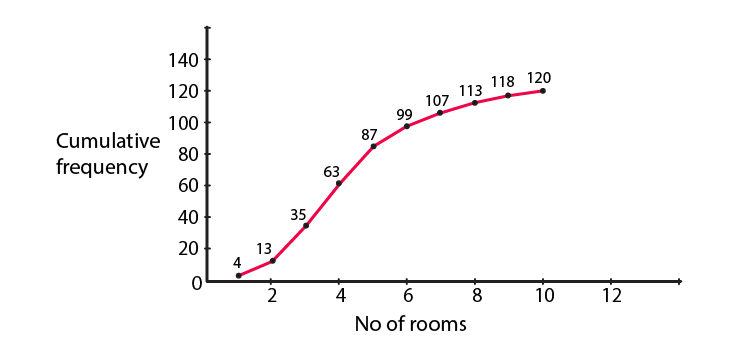2. The marks scored by 750 students in an examination are given in the form of a frequency distribution table.

 Marks No. of Students 600 – 640 16 640 – 680 45 680 – 720 156 720 – 760 284 760 – 800 172 800 – 840 59 840 – 880 18

Prepare a cumulative frequency distribution table by less than method and draw an ogive.

Solution:

 Marks No. of Students Marks Less than Cumulative Frequency 600 – 640 16 640 16 640 – 680 45 680 61 680 – 720 156 720 217 720 – 760 284 760 501 760 – 800 172 800 673 800 – 840 59 840 732 840 – 880 18 880 750

Plot the points (640, 16), (680, 61), (720, 217), (760, 501), (800, 673), (840, 732), (880, 750) by taking upper-class limit over the x-axis and cumulative frequency over the y-axis.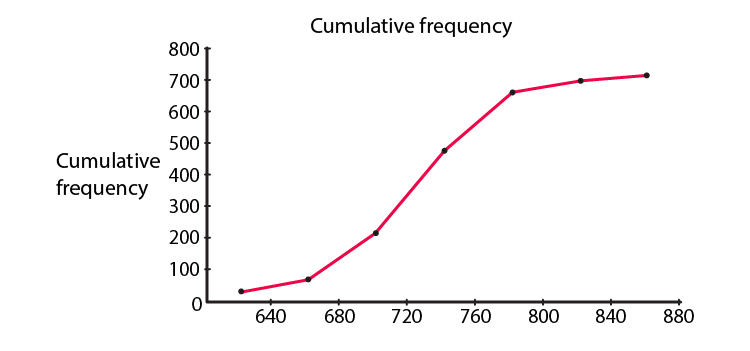3. Draw an Ogive to represent the following frequency distribution.

 Class-interval 0 – 4 5 – 9 10 – 14 15 – 19 20 – 24 No. of students 2 6 10 5 3

Solution:

Since the given frequency distribution is not continuous, we will have to first make it continuous and then prepare the cumulative frequency.

 Class interval No. of Students Less than Cumulative frequency 0.5 – 4.5 2 4.5 2 4.5 – 9.5 6 9.5 8 9.5 – 14.5 10 14.5 18 14.5 – 19.5 5 19.5 23 19.5 – 24.5 3 24.5 26

Plot the points (4.5, 2), (9.5, 8), (14.5, 18), (19.5, 23), (24.5, 26) by taking the upper-class limit over the x-axis and cumulative frequency over the y-axis.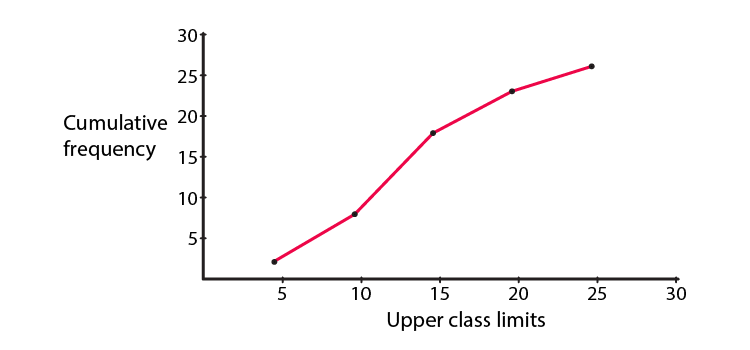4. The monthly profits (in Rs) of 100 shops are distributed as follows:

 Profit per shop No of shops: 0 – 50 12 50 – 100 18 100 – 150 27 150 – 200 20 200 – 250 17 250 – 300 6

Draw the frequency polygon for it.

Solution:

Doing for the less than method, we have

 Profit per shop Mid-value No. of shops Less than 0 0 0 Less than 0 – 50 25 12 Less than 50 – 100 75 18 Less than 100 – 150 125 27 Less than 150 – 200 175 20 Less than 200 – 250 225 17 Less than 250 – 300 275 6 Above 300 300 0

By plotting the respective coordinates, we can get the frequency polygon.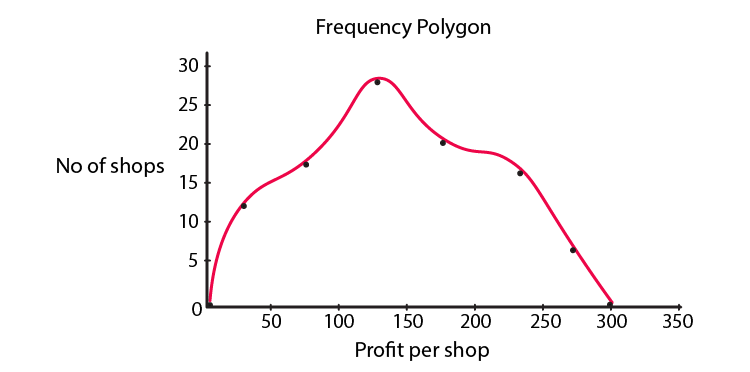5. The following distribution gives the daily income of 50 workers of a factory:

 Daily income (in Rs): No of workers: 100 – 120 12 120 – 140 14 140 – 160 8 160 – 180 6 180 – 200 10

Convert the above distribution to a ‘less than’ type cumulative frequency distribution and draw its ogive.

Solution:

Firstly, we prepare the cumulative frequency table by less than method as given below.

 Daily income Cumulative frequency Less than 120 12 Less than 140 26 Less than 160 34 Less than 180 40 Less than 200 50

Now, we mark on the x-axis upper-class limit and y-axis cumulative frequencies. Thus, we plot point (120, 12), (140, 26), (160, 34), (180, 40), (200, 50).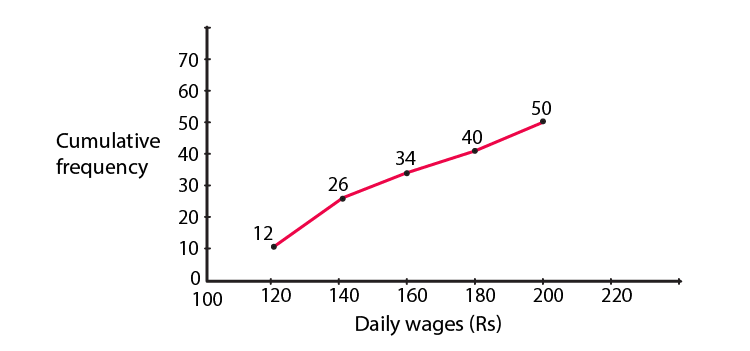## Frequently Asked Questions on RD Sharma Solutions for Class 10 Maths Chapter 7

### How are RD Sharma Solutions for Class 10 Maths Chapter 7 helpful for board exams?

RD Sharma Solutions for Class 10 Maths Chapter 7 provide answers with detailed descriptions, as per term limits prescribed by the Board. Solving these solutions will provide excellent practice for the students so they can finish the paper on time. So, it’s clear that the RD Sharma Solutions for Class 10 Maths Chapter 7 are essential to score high in examinations. Students can get acquainted with writing exams and will be able to face exams more confidently.

### Mention the topics that are covered in RD Sharma Solutions for Class 10 Maths Chapter 7.

The topics that are covered in RD Sharma Solutions for Class 10 Maths Chapter 7 are the the techniques for finding the mean, median and mode of grouped data. It also includes the concept of a cumulative frequency graph of a frequency distribution. Statistics is the study of the collection, analysis, interpretation, presentation and organisation of data. In other words, it is a mathematical discipline to collect and summarise data.

### Is it necessary to practise all the exercises presented in Chapter 7 of RD Sharma Solutions for Class 10 Maths?

Yes, it is compulsory to practise all the exercises presented in Chapter 7 of RD Sharma Solutions for Class 10 Maths. This is because all exercises contain numerous questions to solve, which may come in their finals. This makes them more confident towards the syllabus they have. The solutions are designed based on the latest CBSE syllabus and exam pattern. Understanding the concepts before solving the exercise-wise problems is very important to obtain a better score in the exam. The questions presented in this have been solved by BYJU’S experts in Maths, and these solutions guide students to study well and solve lengthy problems in a shorter duration.